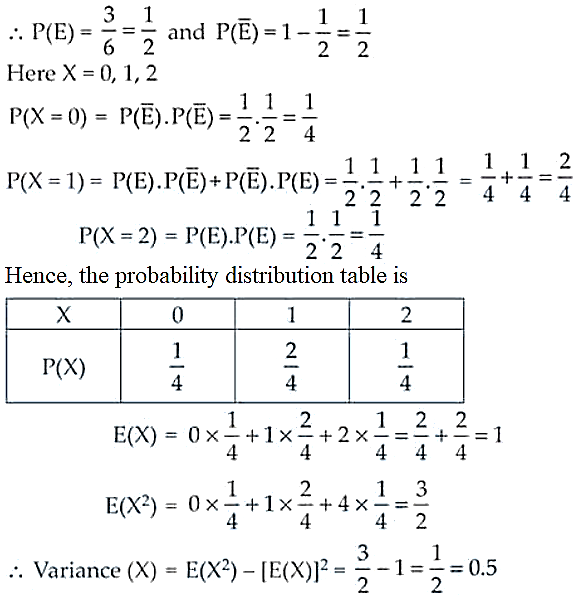# NCERT Exemplar Solutions for Class 12 Maths Chapter 13 Probability

The NCERT Exemplar is the best resource for learning advanced concepts in an easy way and also for practising and preparing for examinations. These exemplar textbooks are for Classes 6 to 12 for the subjects Mathematics and Science based on the NCERT syllabus. For guidance in solving the exemplar problems, students can access NCERT Exemplar Solutions. The solutions are created by subject experts at BYJU’S, according to the latest CBSE guidelines.

Probability is the last chapter of the NCERT Exemplar Solutions for Class 12 Mathematics. This chapter has exemplar problems based on conditional probability and its properties, the multiplication theorem on probability independent events, Bayes’ theorem, the partition of a sample space, the theorem of total probability, random variables and probability distributions, mean and variance of a random variable, and Bernoulli trials and binomial distribution. Students can clarify any doubts that arise while solving problems from the chapter by accessing the solutions PDF of NCERT Exemplar Solutions for Class 12 Maths Chapter 13 Probability from the link given below.

## Download the PDF of NCERT Exemplar Solutions for Class 12 Maths Chapter 13 Probability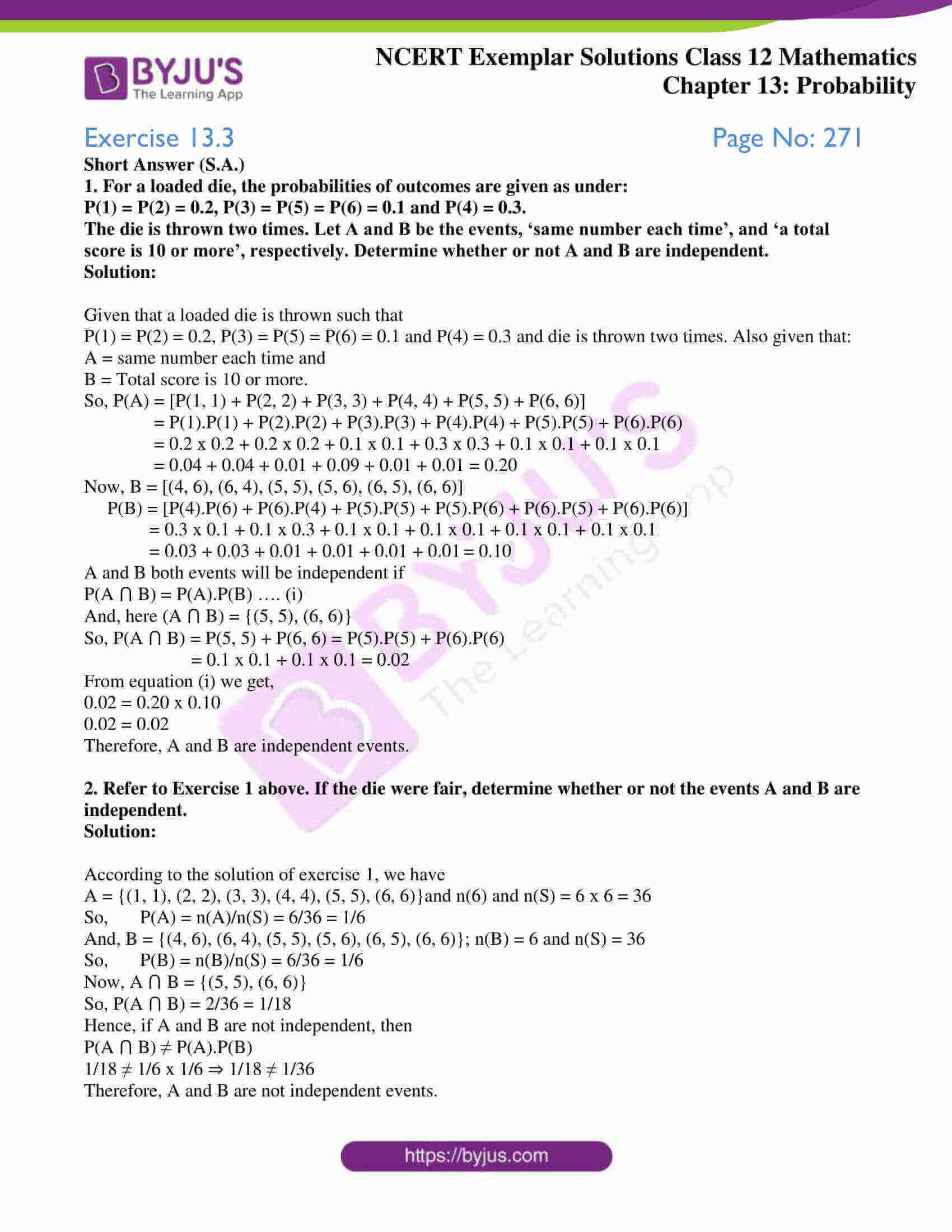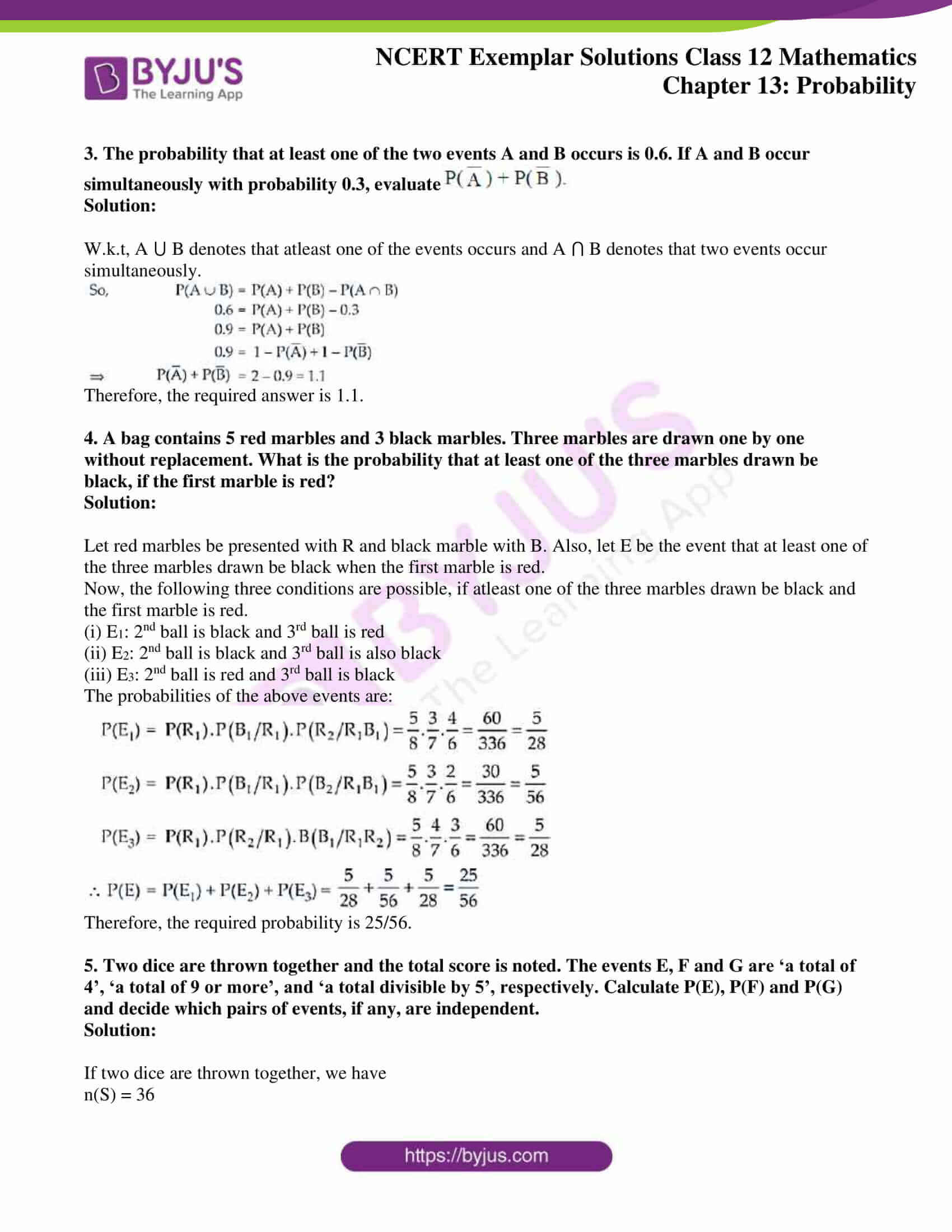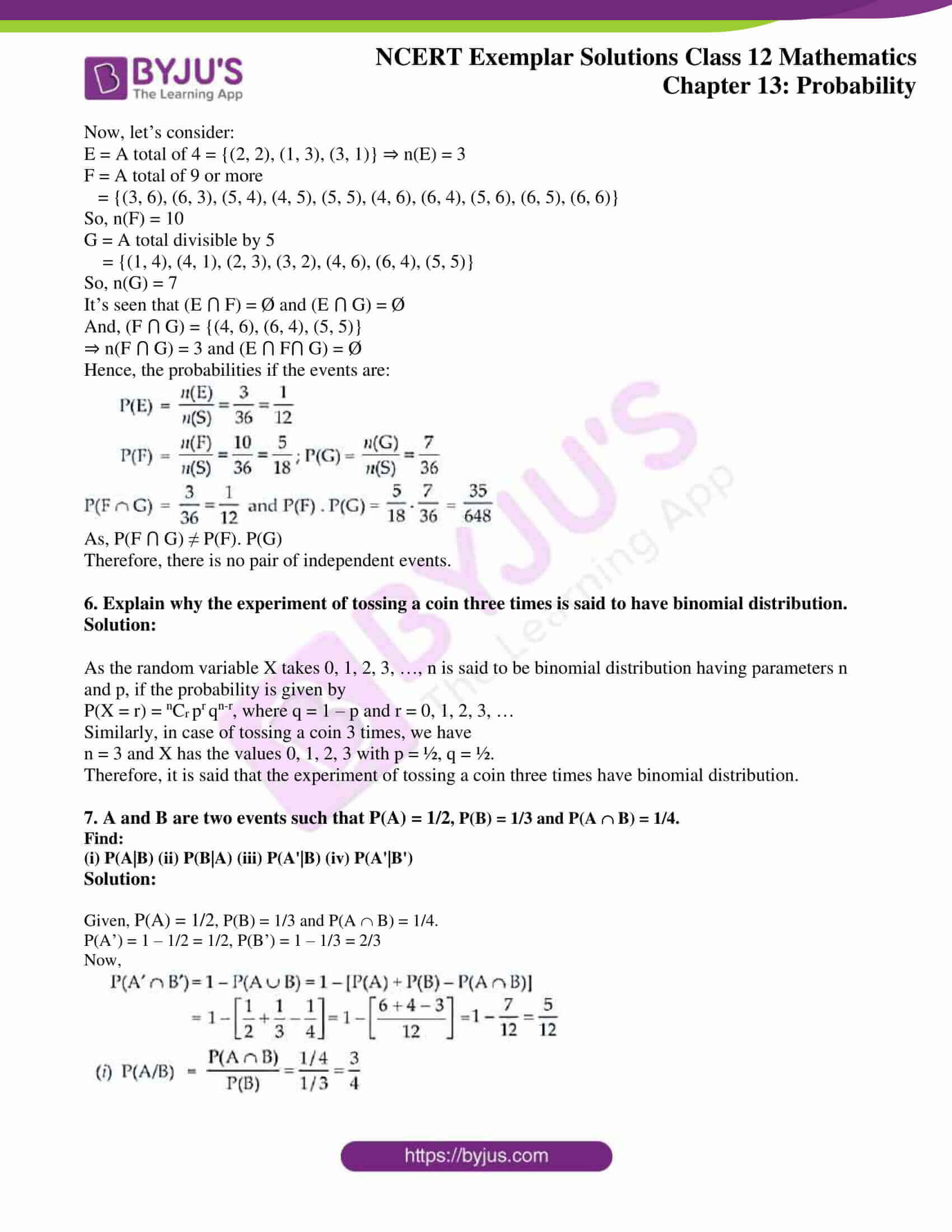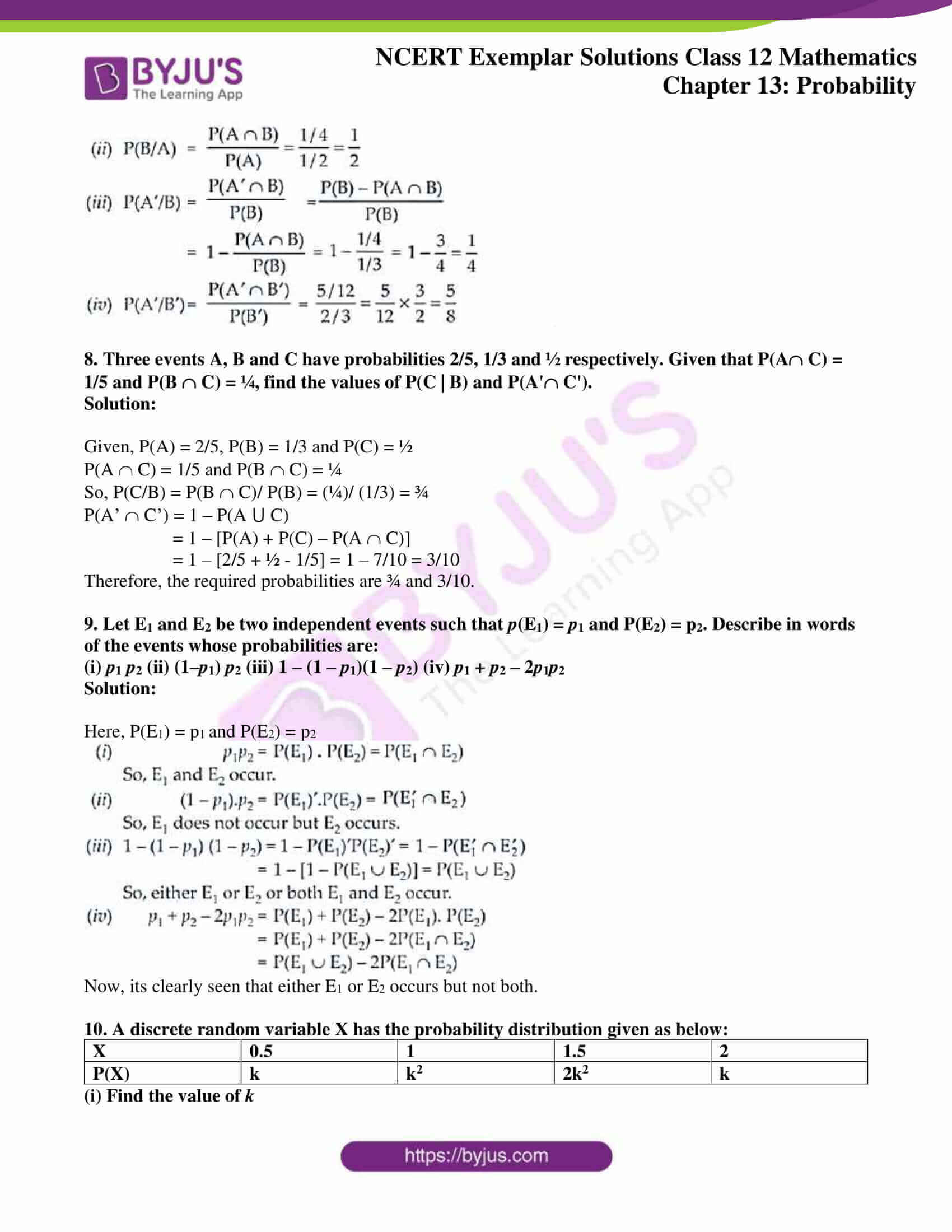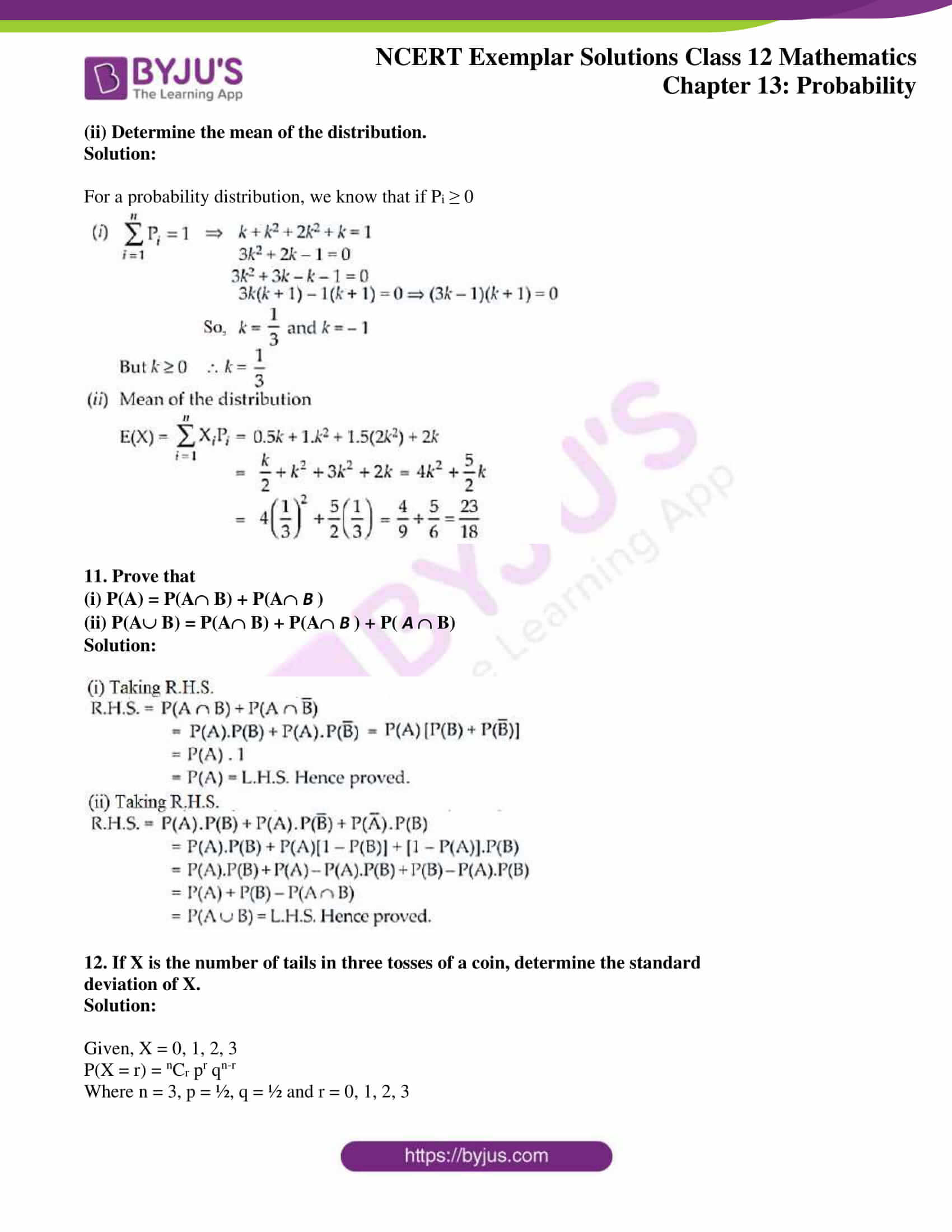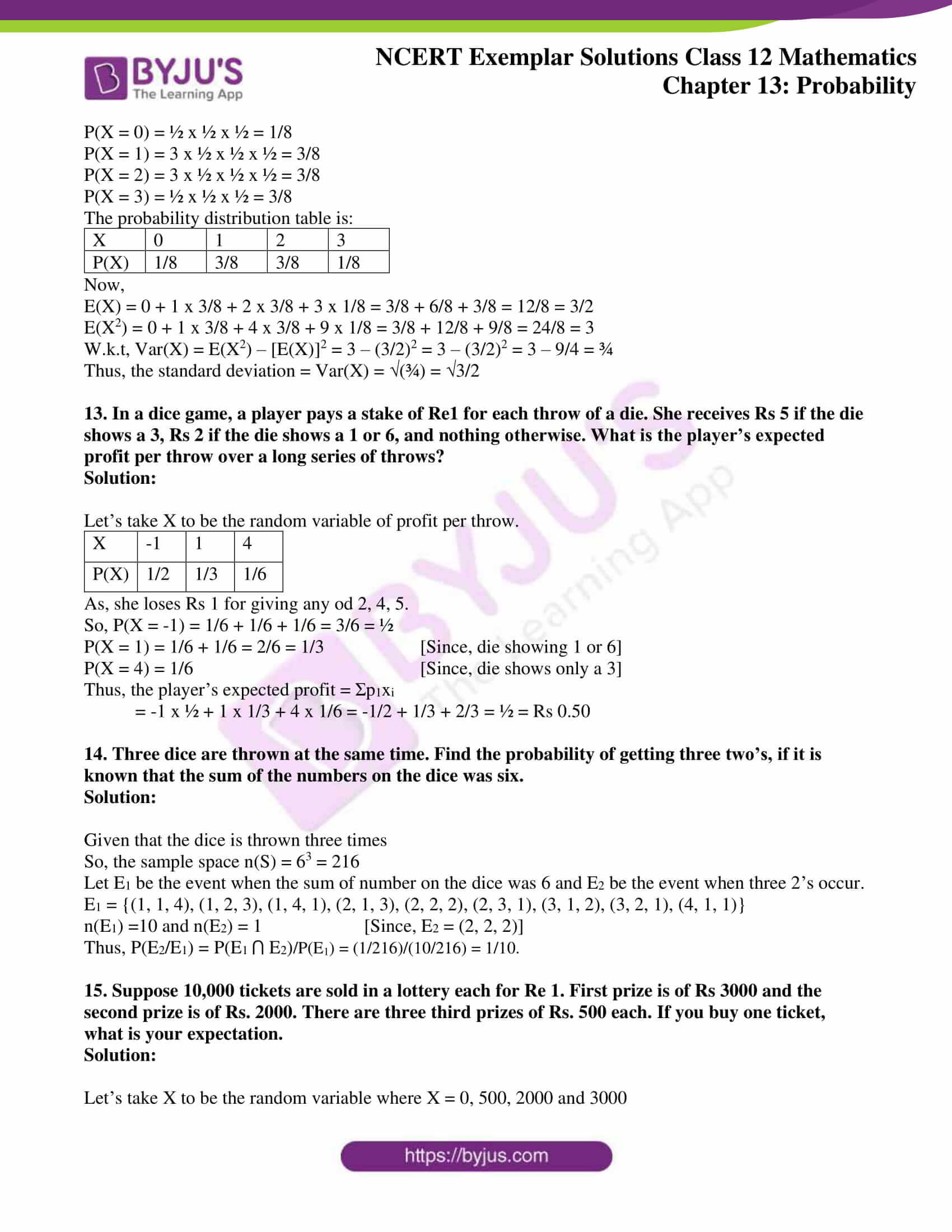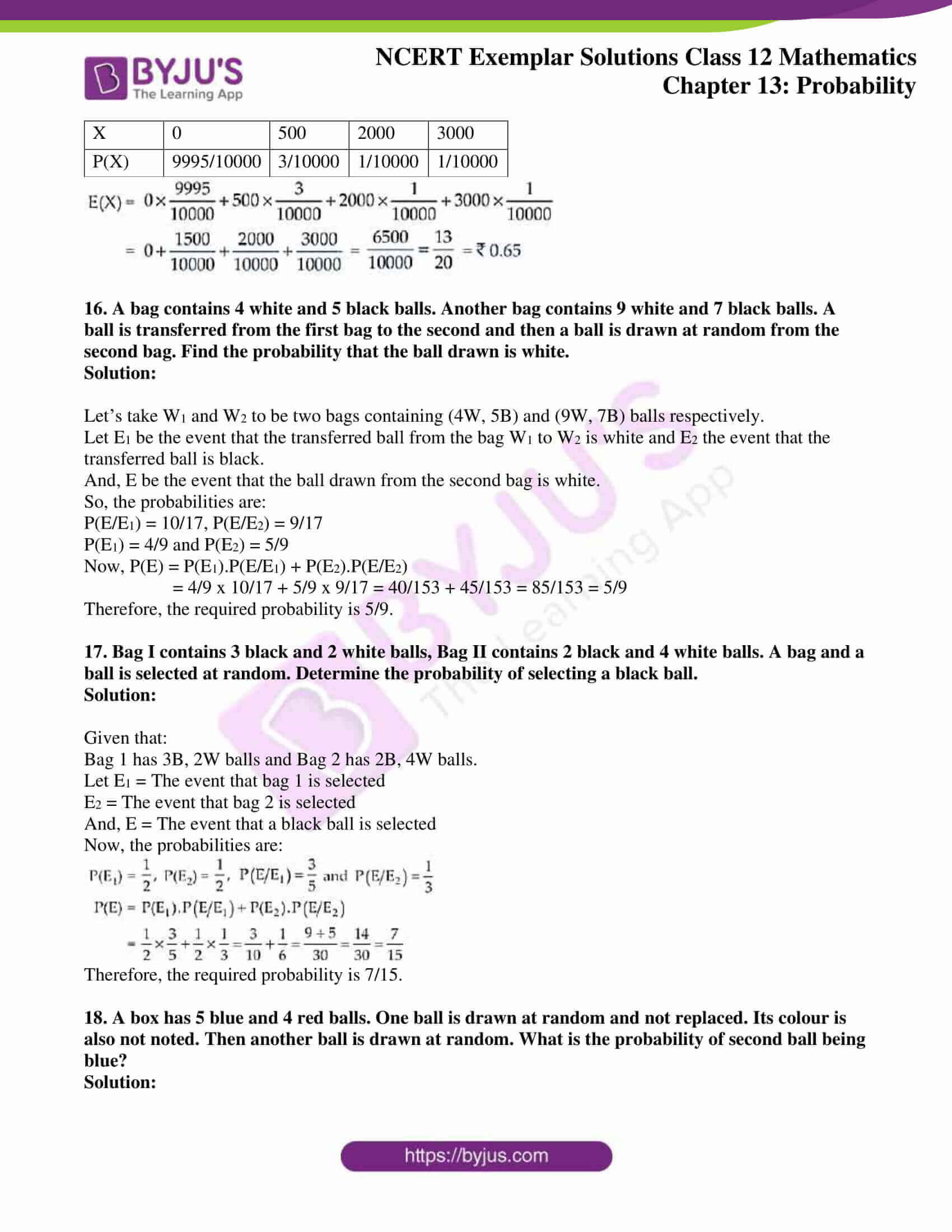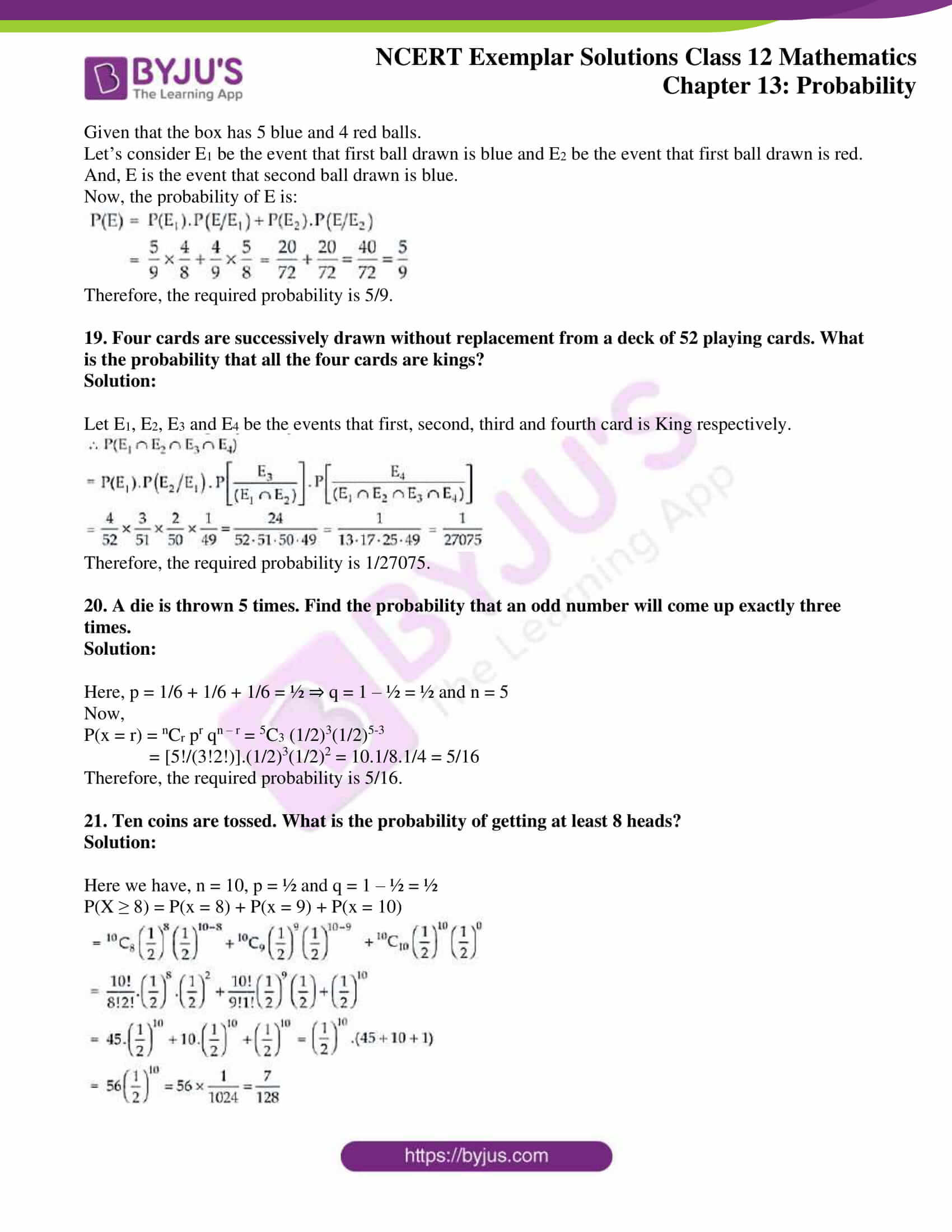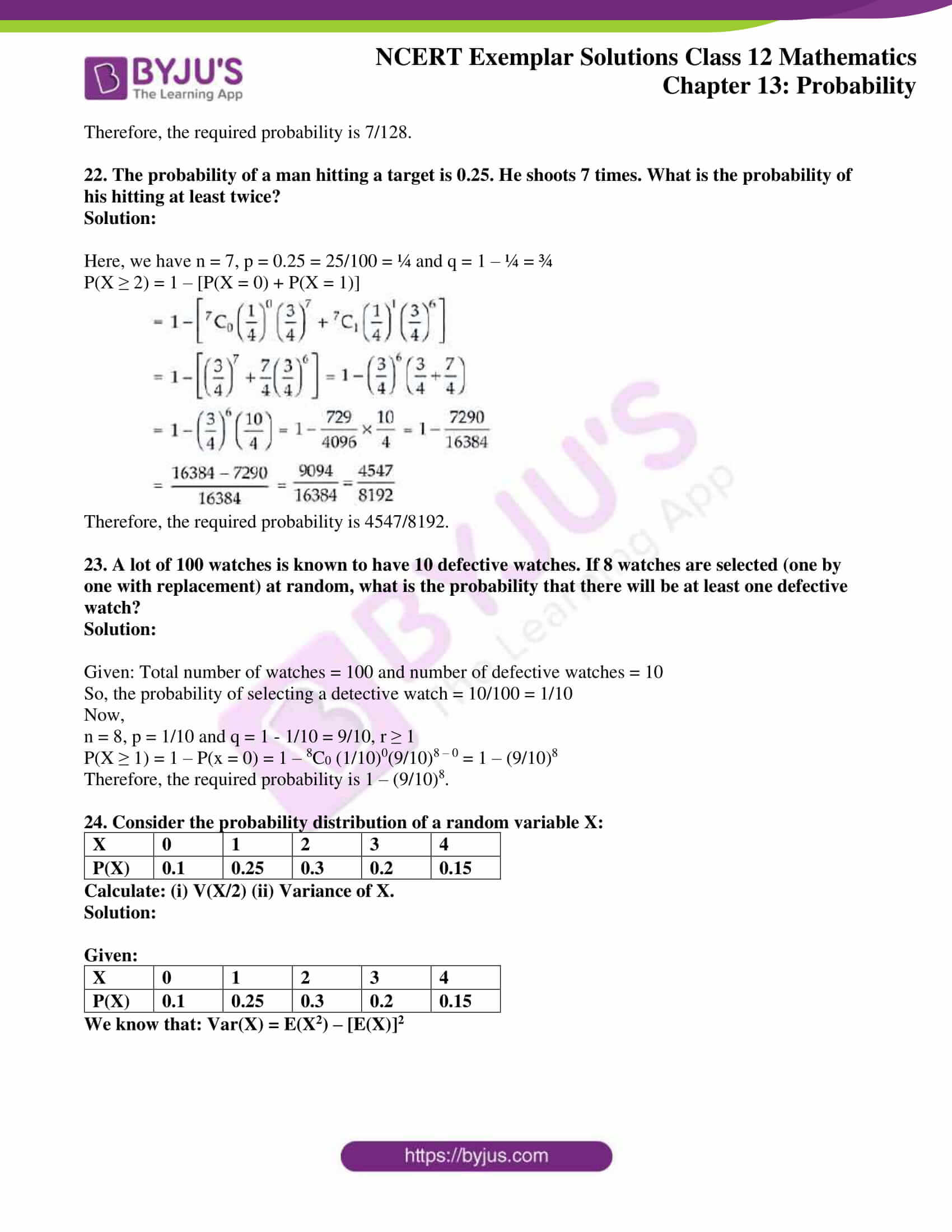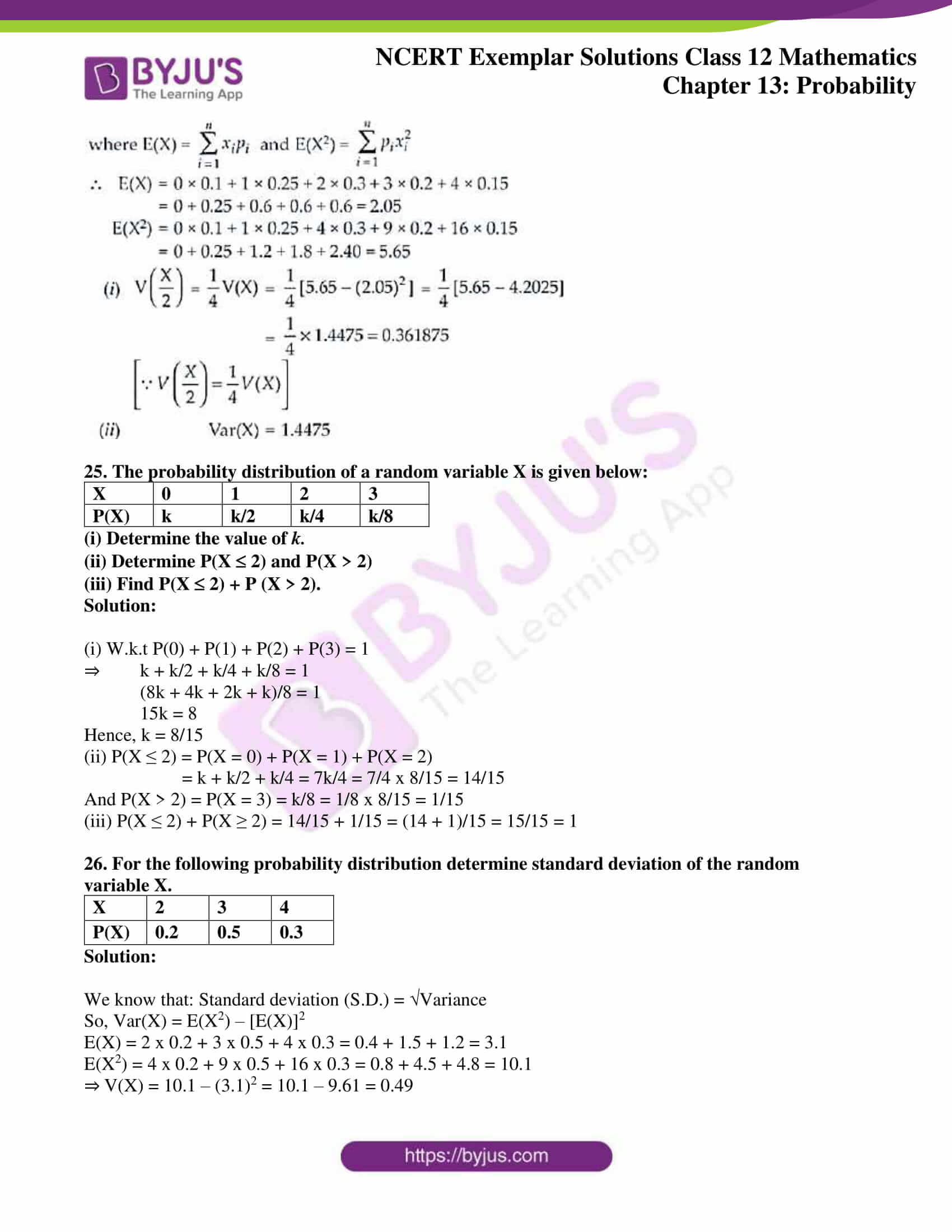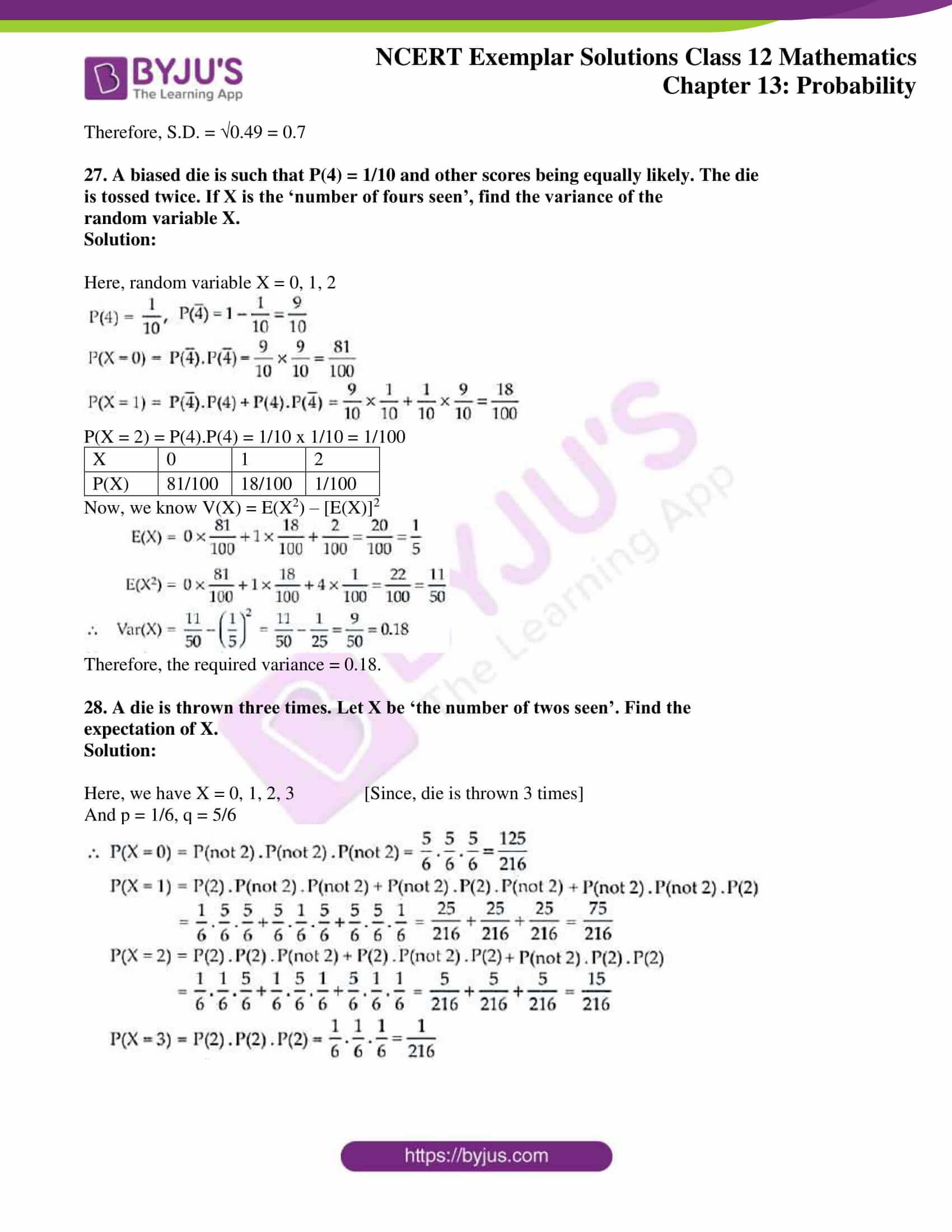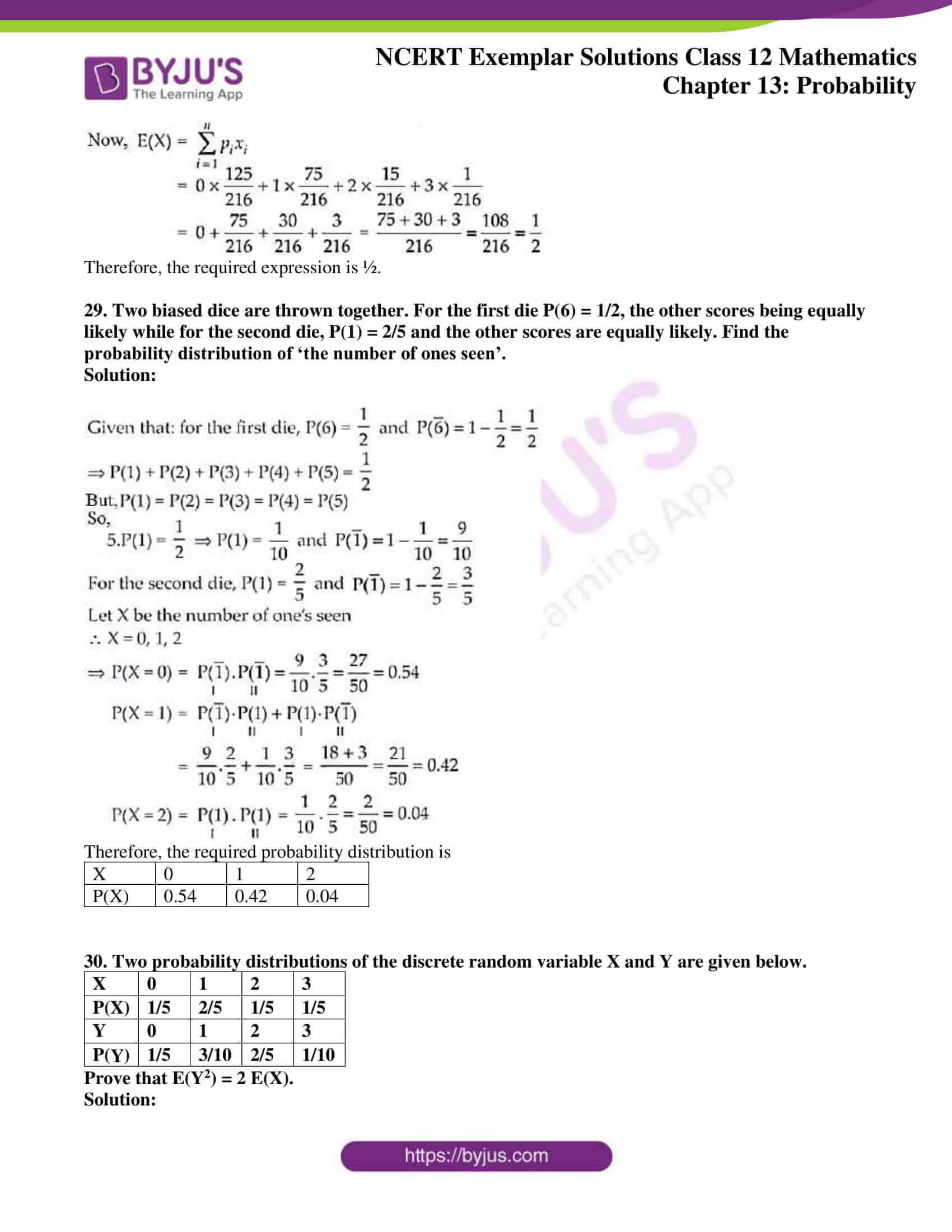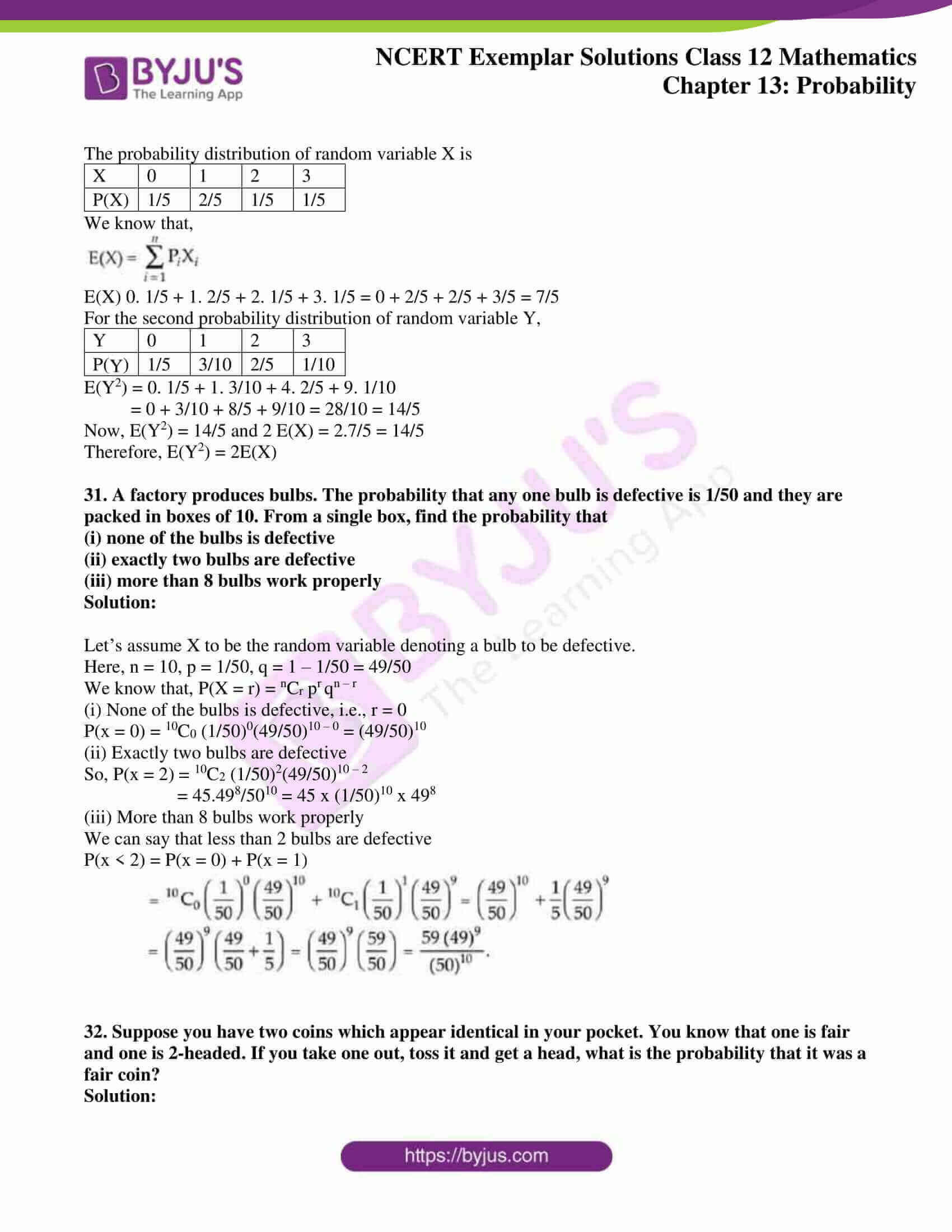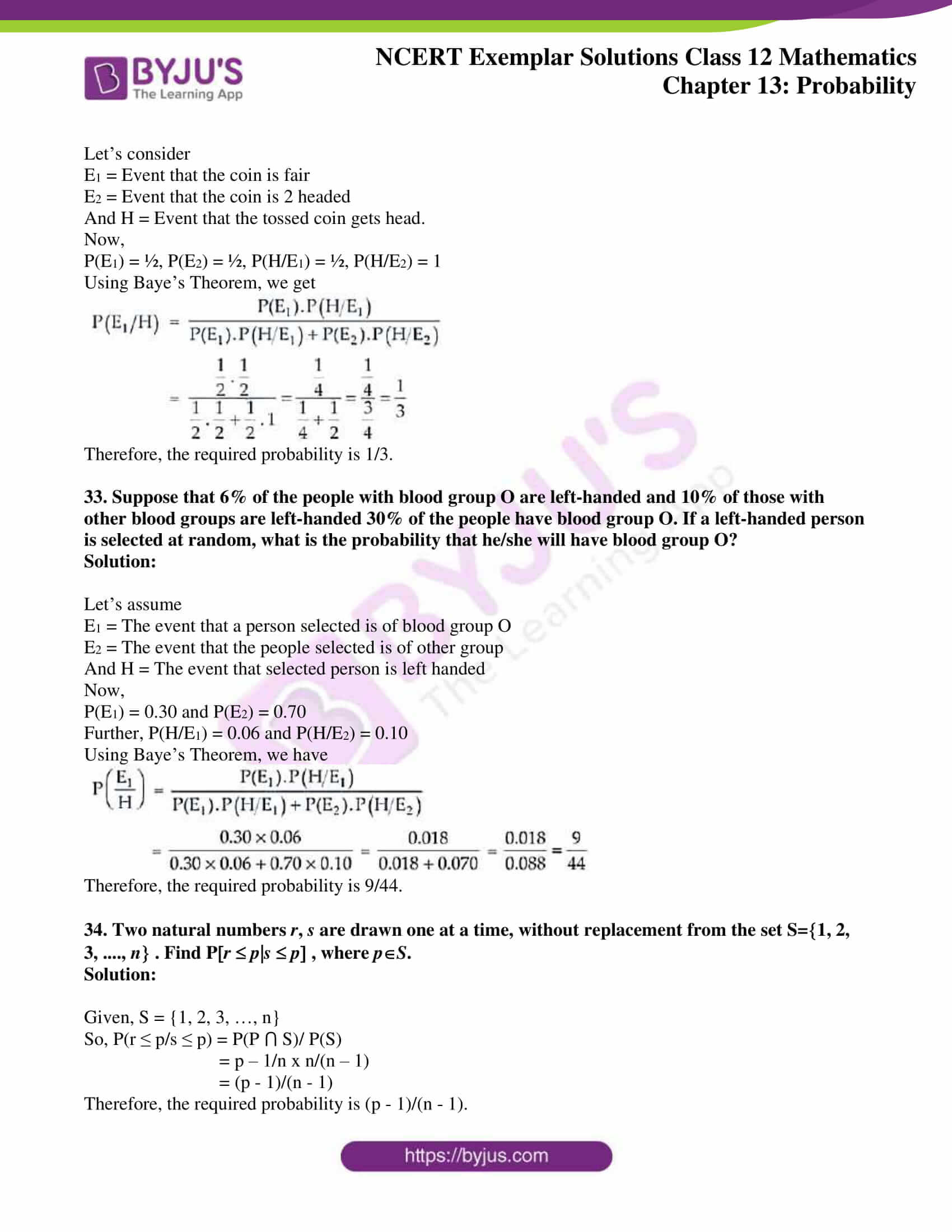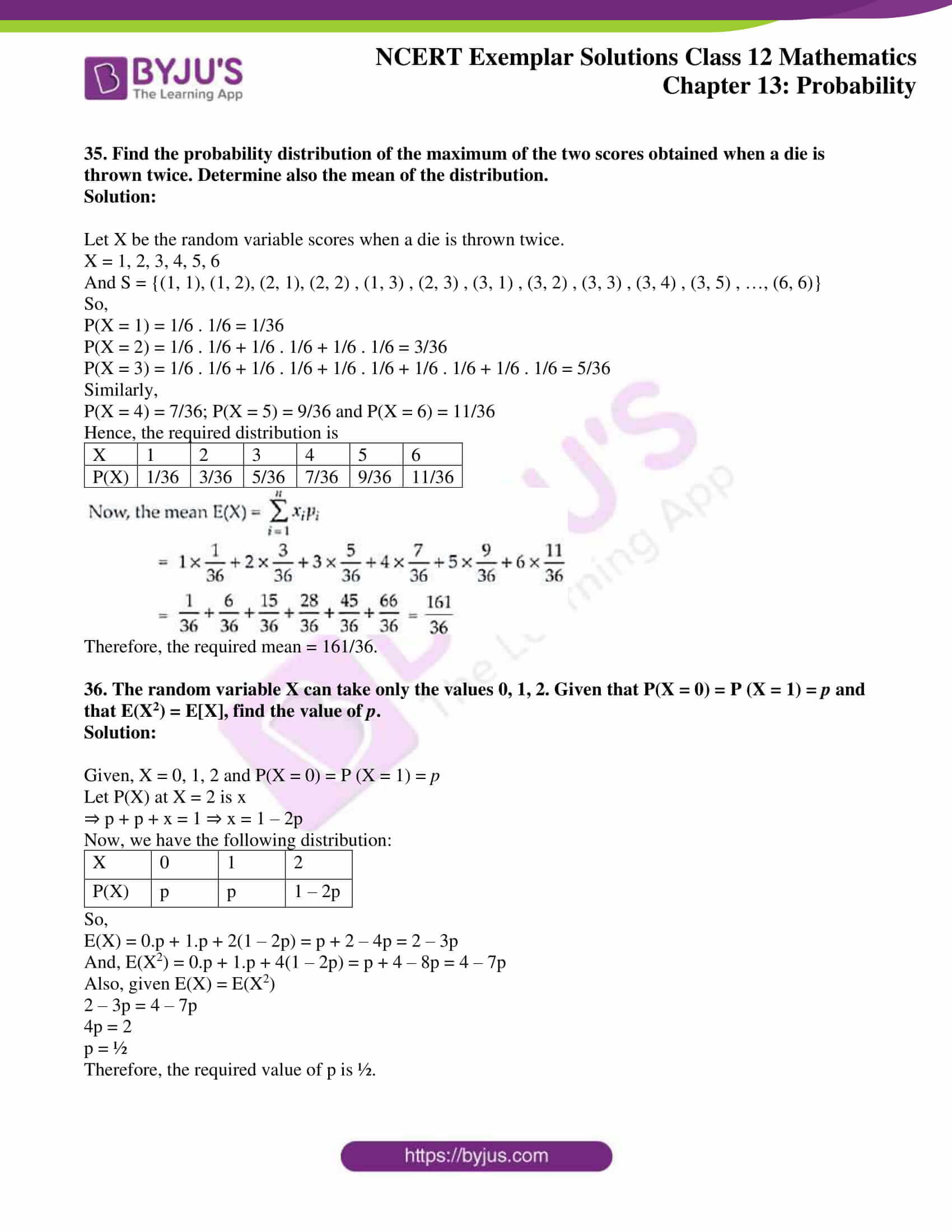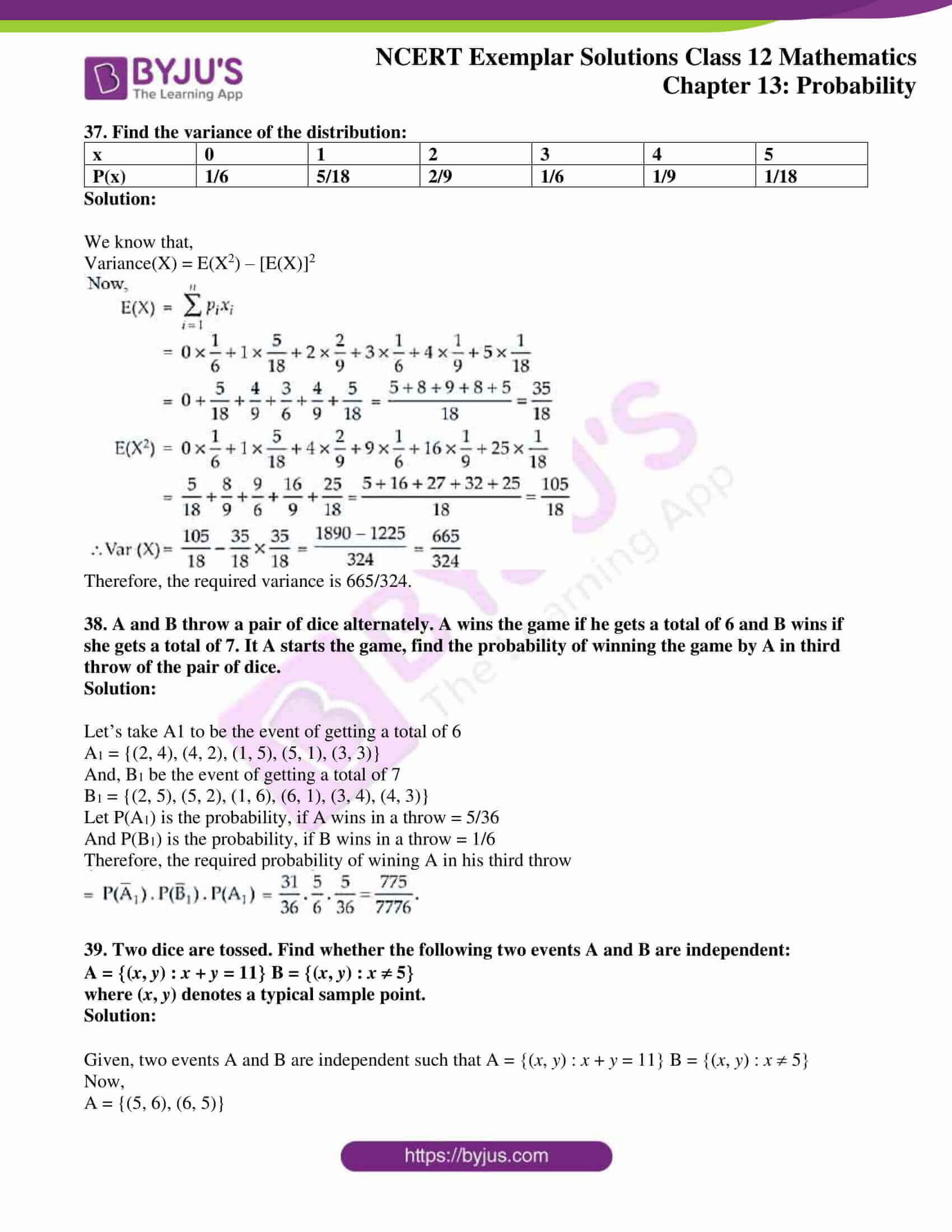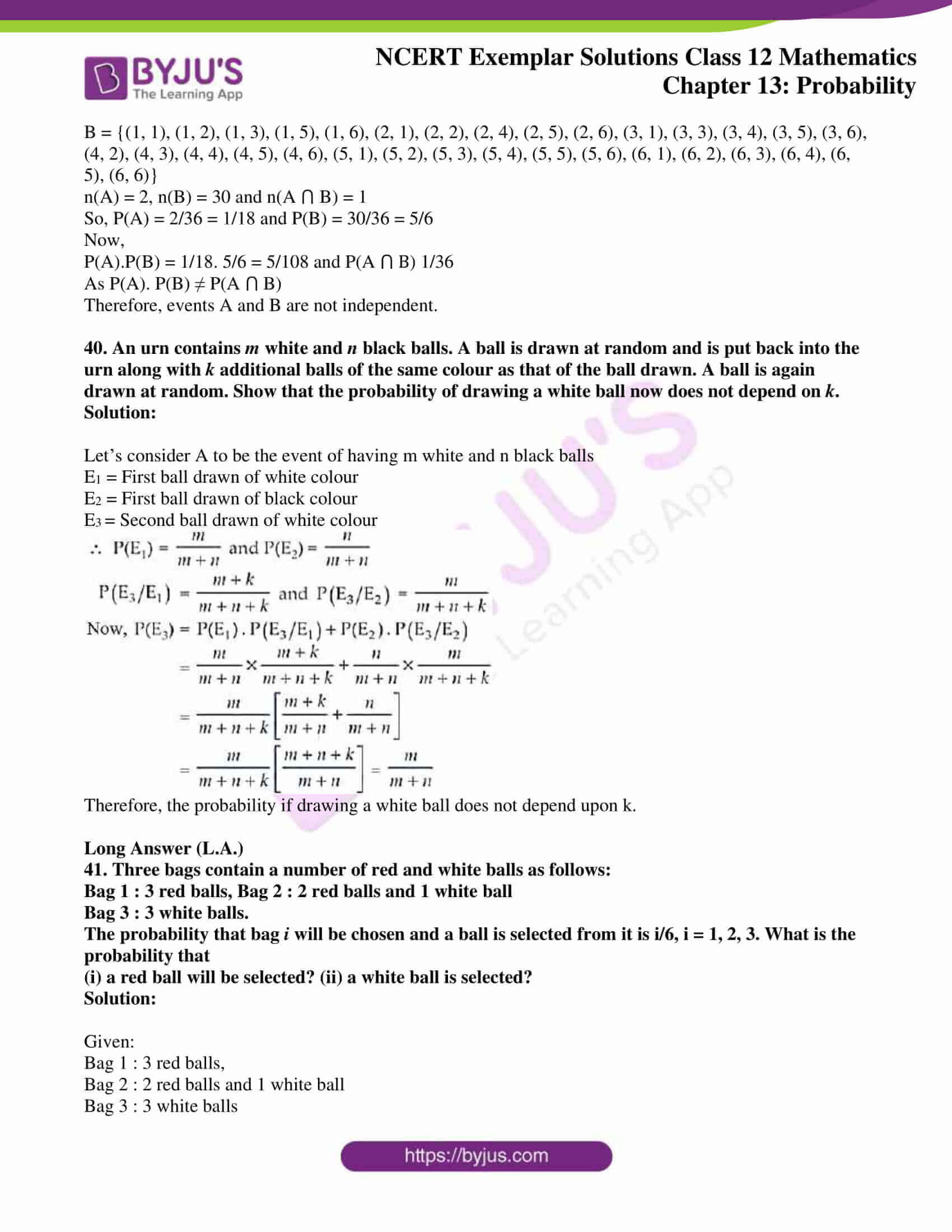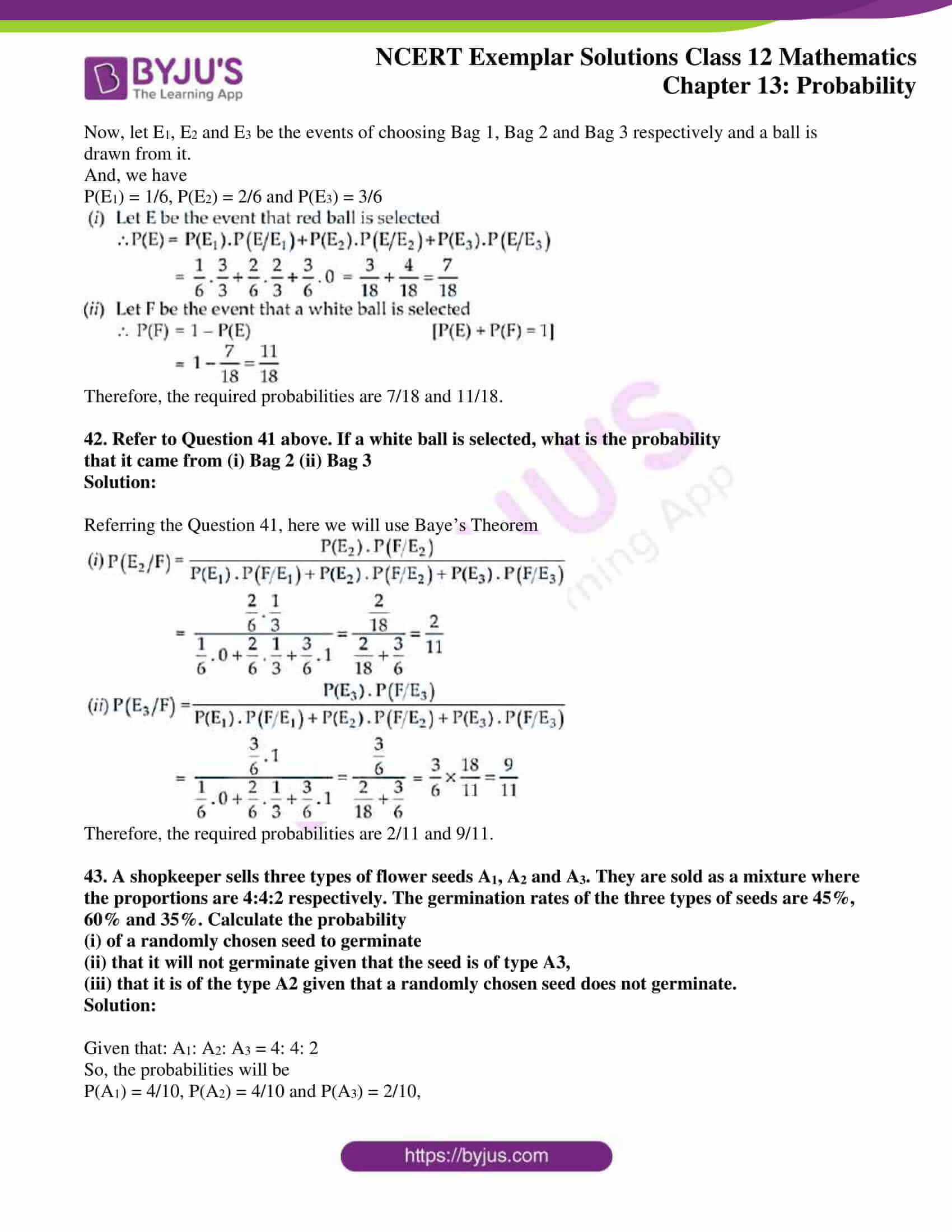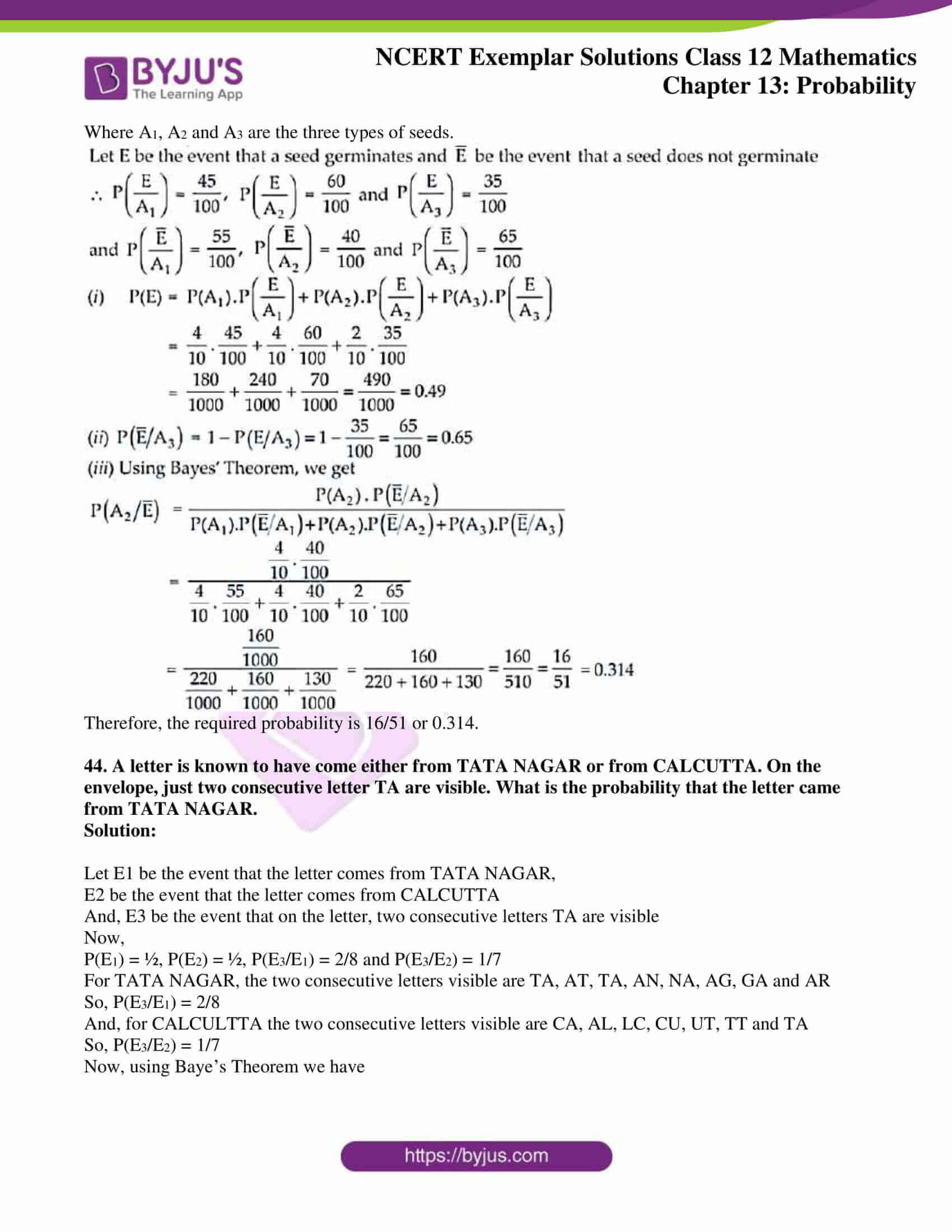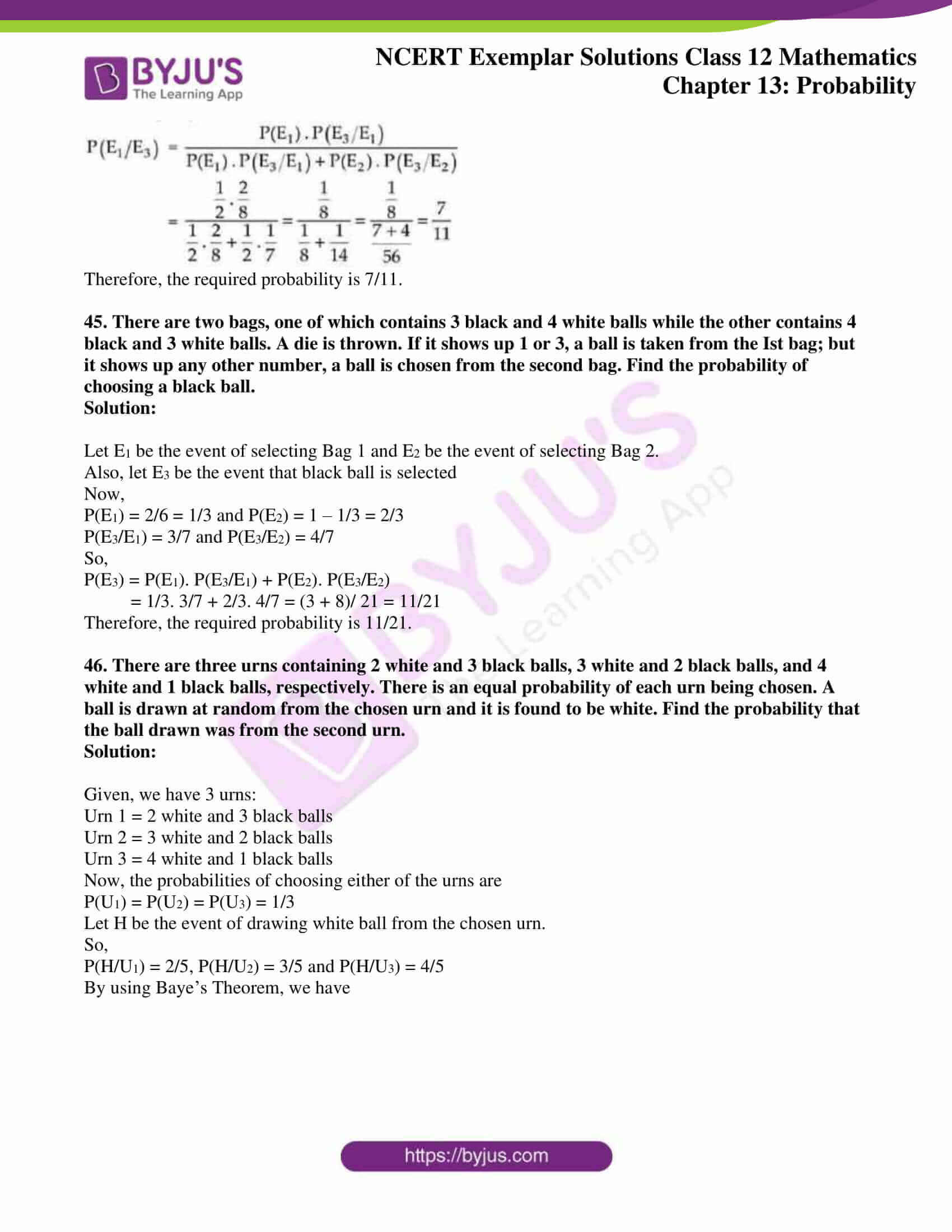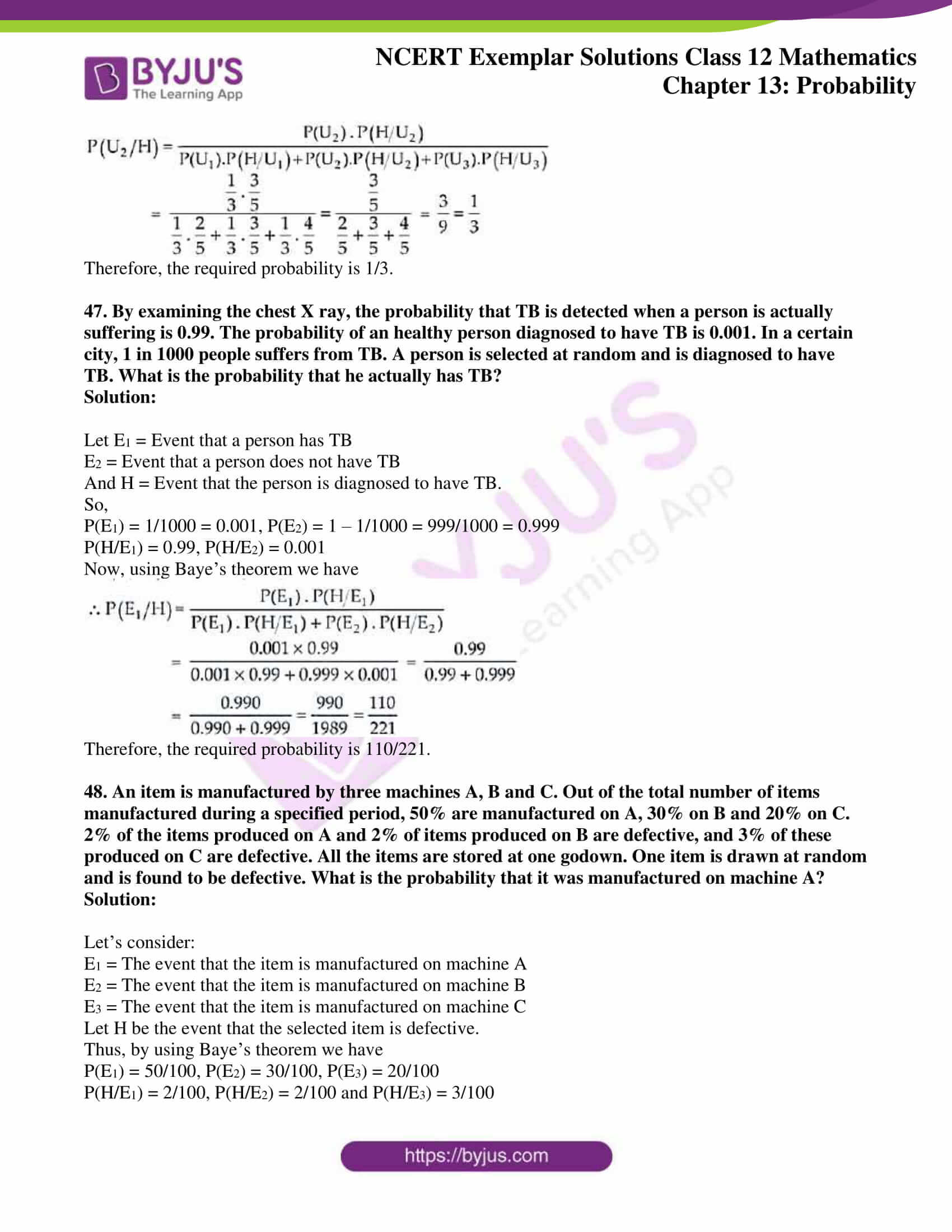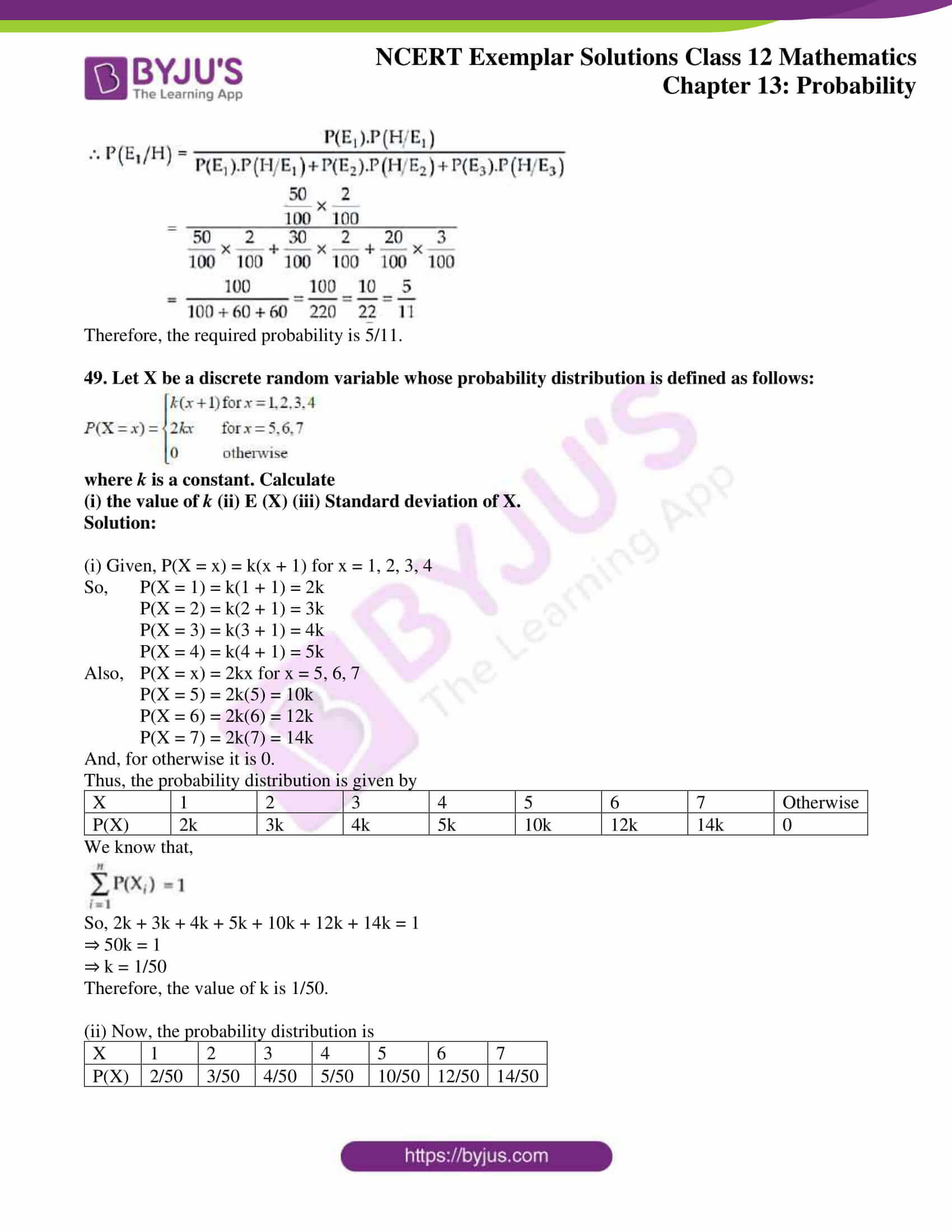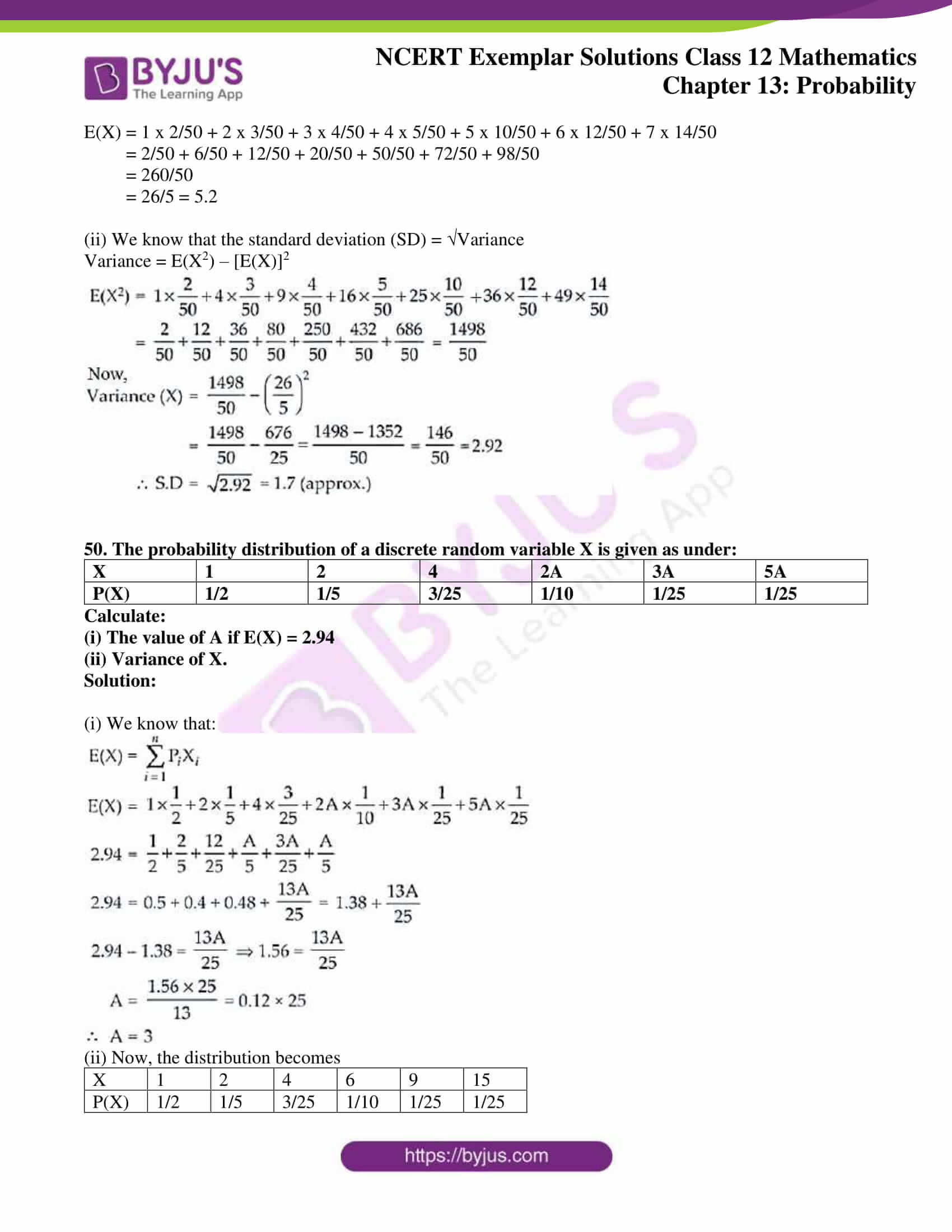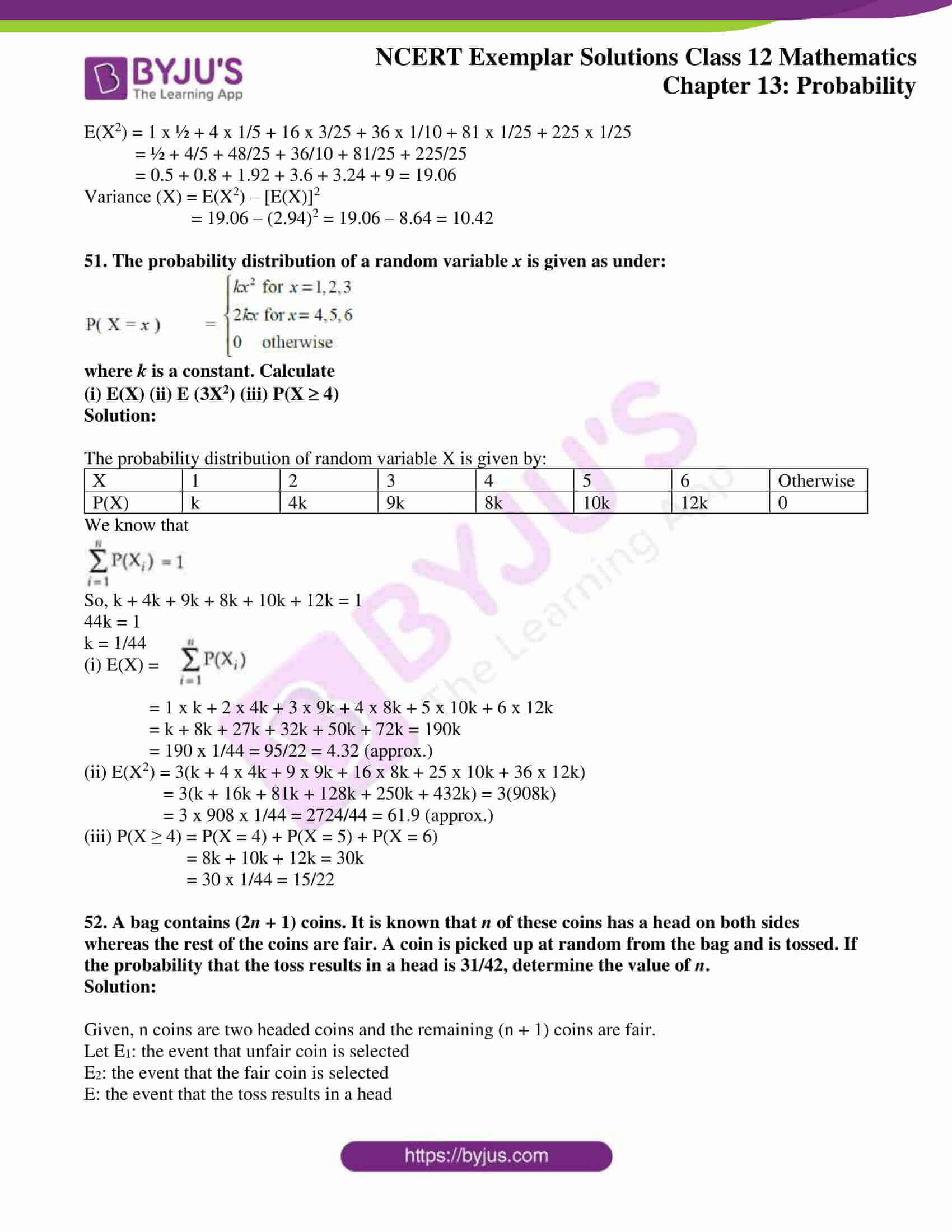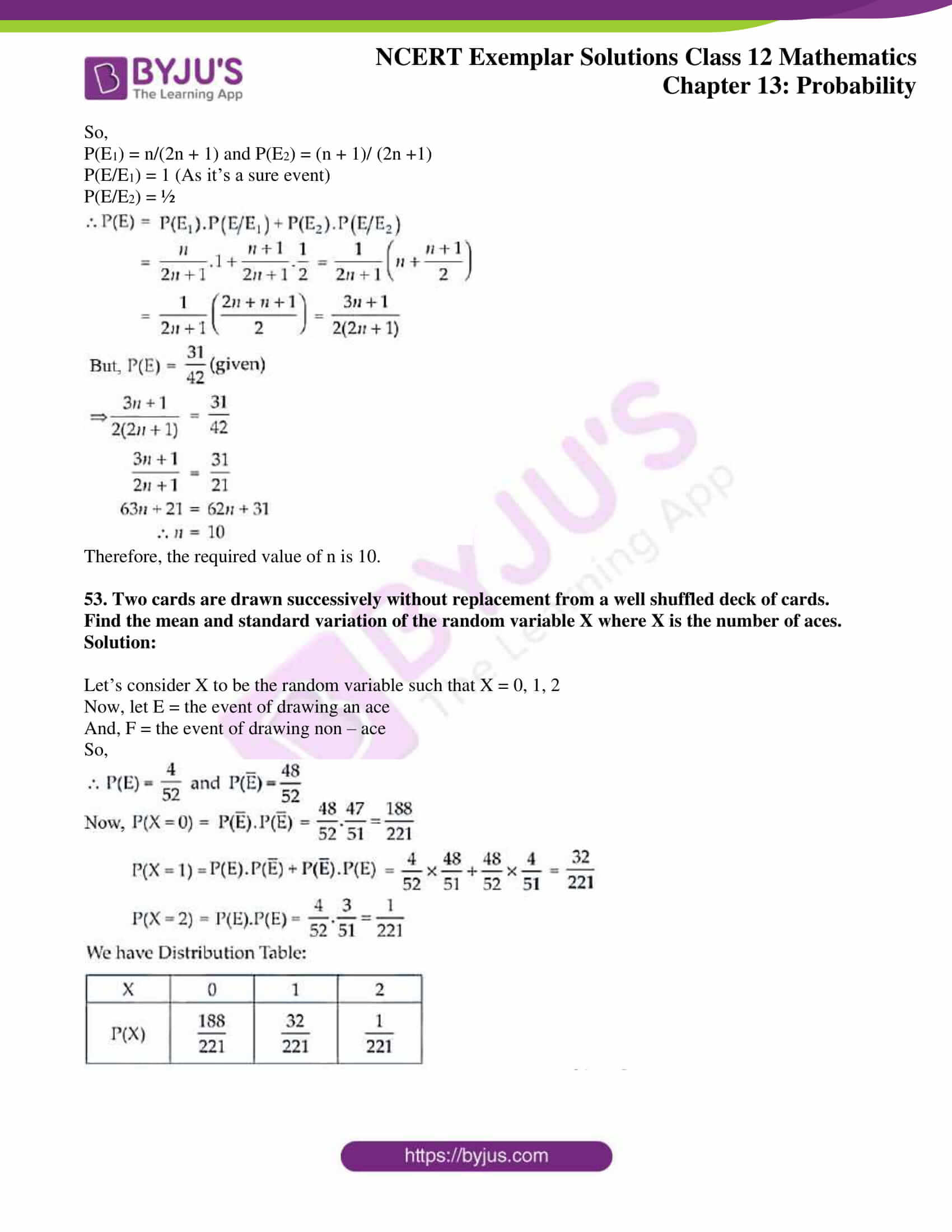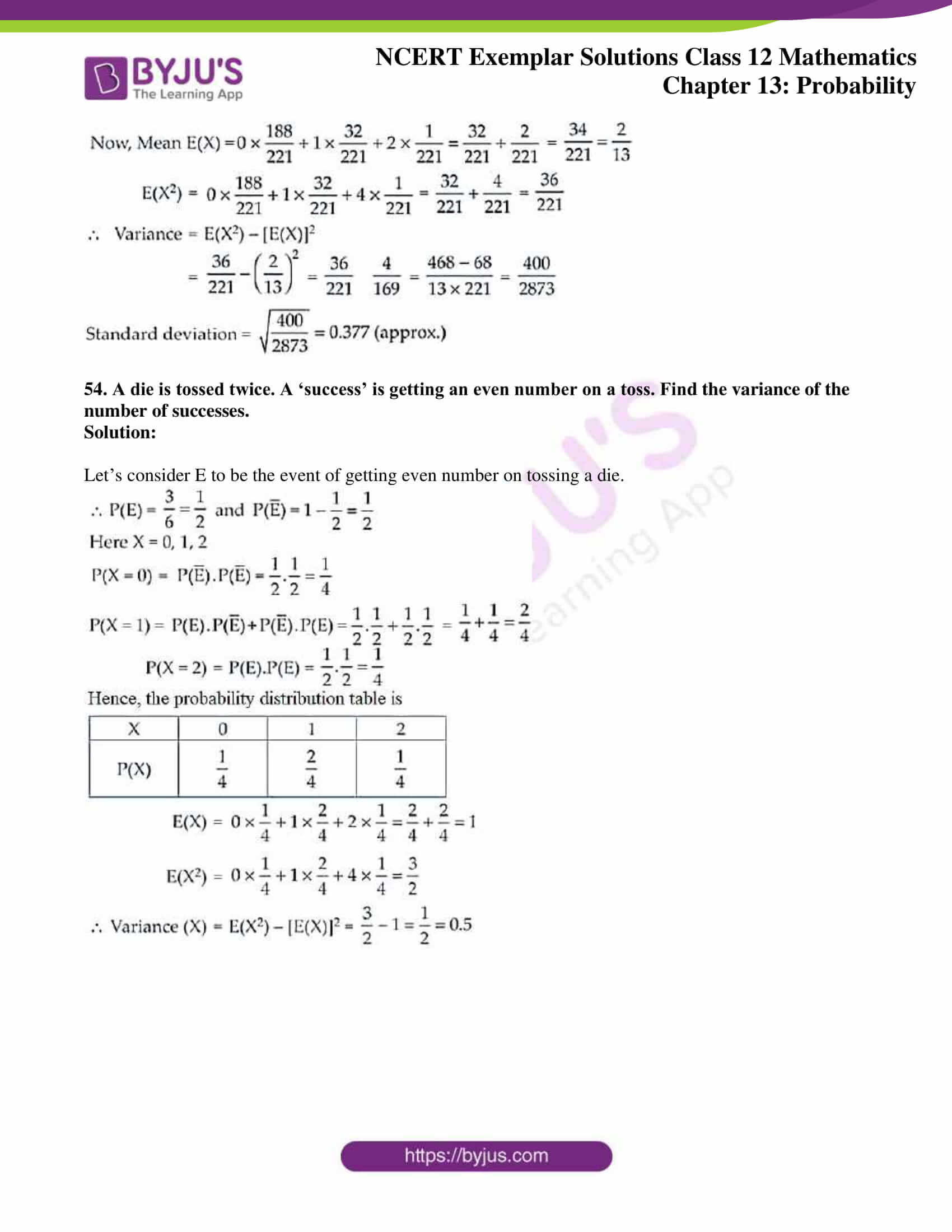### Access Answers to NCERT Exemplar Solutions for Class 12 Maths Chapter 13 Probability

Exercise 13.3 Page No: 271

1. For a loaded die, the probabilities of outcomes are given as under:

P(1) = P(2) = 0.2, P(3) = P(5) = P(6) = 0.1 and P(4) = 0.3.

The die is thrown two times. Let A and B be the events, ‘same number each time’, and ‘a total score is 10 or more’, respectively. Determine whether or not A and B are independent.

Solution:

Given that a loaded die is thrown such that

P(1) = P(2) = 0.2, P(3) = P(5) = P(6) = 0.1 and P(4) = 0.3 and die is thrown two times. Also given that:

A = same number each time and

B = Total score is 10 or more.

So, P(A) = [P(1, 1) + P(2, 2) + P(3, 3) + P(4, 4) + P(5, 5) + P(6, 6)]

= P(1).P(1) + P(2).P(2) + P(3).P(3) + P(4).P(4) + P(5).P(5) + P(6).P(6)

= 0.2 x 0.2 + 0.2 x 0.2 + 0.1 x 0.1 + 0.3 x 0.3 + 0.1 x 0.1 + 0.1 x 0.1

= 0.04 + 0.04 + 0.01 + 0.09 + 0.01 + 0.01 = 0.20

Now, B = [(4, 6), (6, 4), (5, 5), (5, 6), (6, 5), (6, 6)]

P(B) = [P(4).P(6) + P(6).P(4) + P(5).P(5) + P(5).P(6) + P(6).P(5) + P(6).P(6)]

= 0.3 x 0.1 + 0.1 x 0.3 + 0.1 x 0.1 + 0.1 x 0.1 + 0.1 x 0.1 + 0.1 x 0.1

= 0.03 + 0.03 + 0.01 + 0.01 + 0.01 + 0.01 = 0.10

A and B both events will be independent if

P(A ⋂ B) = P(A).P(B) …. (i)

And, here (A ⋂ B) = {(5, 5), (6, 6)}

So, P(A ⋂ B) = P(5, 5) + P(6, 6) = P(5).P(5) + P(6).P(6)

= 0.1 x 0.1 + 0.1 x 0.1 = 0.02

From equation (i) we get,

0.02 = 0.20 x 0.10

0.02 = 0.02

Therefore, A and B are independent events.

2. Refer to Exercise 1 above. If the die were fair, determine whether or not the events A and B are independent.

Solution:

According to the solution of exercise 1, we have

A = {(1, 1), (2, 2), (3, 3), (4, 4), (5, 5), (6, 6)}and n(6) and n(S) = 6 x 6 = 36

So, P(A) = n(A)/n(S) = 6/36 = 1/6

And, B = {(4, 6), (6, 4), (5, 5), (5, 6), (6, 5), (6, 6)}; n(B) = 6 and n(S) = 36

So, P(B) = n(B)/n(S) = 6/36 = 1/6

Now, A ⋂ B = {(5, 5), (6, 6)}

So, P(A ⋂ B) = 2/36 = 1/18

Hence, if A and B are not independent, then

P(A ⋂ B) ≠ P(A).P(B)

1/18 ≠ 1/6 x 1/6 ⇒ 1/18 ≠ 1/36

Therefore, A and B are not independent events.

3. The probability that at least one of the two events A and B occurs is 0.6. If A and B occur simultaneously with probability 0.3, evaluate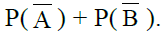Solution:

W.k.t, A ⋃ B denotes that atleast one of the events occurs and A ⋂ B denotes that two events occur simultaneously.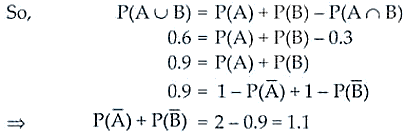Therefore, the required answer is 1.1.

4. A bag contains 5 red marbles and 3 black marbles. Three marbles are drawn one by one without replacement. What is the probability that at least one of the three marbles drawn be black, if the first marble is red?

Solution:

Let red marbles be presented with R and black marble with B. Also, let E be the event that at least one of the three marbles drawn be black when the first marble is red.

Now, the following three conditions are possible, if atleast one of the three marbles drawn be black and the first marble is red.

(i) E1: 2nd ball is black and 3rd ball is red

(ii) E2: 2nd ball is black and 3rd ball is also black

(iii) E3: 2nd ball is red and 3rd ball is black

The probabilities of the above events are: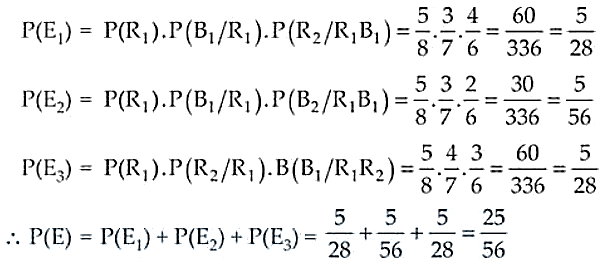Therefore, the required probability is 25/56.

5. Two dice are thrown together and the total score is noted. The events E, F and G are ‘a total of 4’, ‘a total of 9 or more’, and ‘a total divisible by 5’, respectively. Calculate P(E), P(F) and P(G) and decide which pairs of events, if any, are independent.

Solution:

If two dice are thrown together, we have

n(S) = 36

Now, let’s consider:

E = A total of 4 = {(2, 2), (1, 3), (3, 1)} ⇒ n(E) = 3

F = A total of 9 or more

= {(3, 6), (6, 3), (5, 4), (4, 5), (5, 5), (4, 6), (6, 4), (5, 6), (6, 5), (6, 6)}

So, n(F) = 10

G = A total divisible by 5

= {(1, 4), (4, 1), (2, 3), (3, 2), (4, 6), (6, 4), (5, 5)}

So, n(G) = 7

It’s seen that (E ⋂ F) = Ø and (E ⋂ G) = Ø

And, (F ⋂ G) = {(4, 6), (6, 4), (5, 5)}

⇒ n(F ⋂ G) = 3 and (E ⋂ F⋂ G) = Ø

Hence, the probabilities if the events are: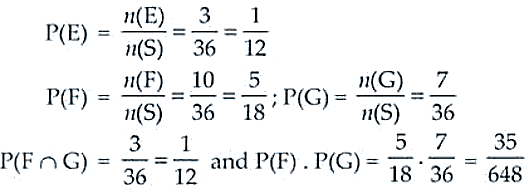As, P(F ⋂ G) ≠ P(F). P(G)

Therefore, there is no pair of independent events.

6. Explain why the experiment of tossing a coin three times is said to have binomial distribution.

Solution:

As the random variable X takes 0, 1, 2, 3, …, n is said to be binomial distribution having parameters n and p, if the probability is given by

P(X = r) = nCr pr qn-r, where q = 1 – p and r = 0, 1, 2, 3, …

Similarly, in case of tossing a coin 3 times, we have

n = 3 and X has the values 0, 1, 2, 3 with p = ½, q = ½.

Therefore, it is said that the experiment of tossing a coin three times have binomial distribution.

7. A and B are two events such that P(A) = 1/2, P(B) = 1/3 and P(A Ç B) = 1/4.

Find:

(i) P(A|B) (ii) P(B|A) (iii) P(A’|B) (iv) P(A’|B’)

Solution:

Given, P(A) = 1/2, P(B) = 1/3 and P(A Ç B) = 1/4.

P(A’) = 1 – 1/2 = 1/2, P(B’) = 1 – 1/3 = 2/3

Now,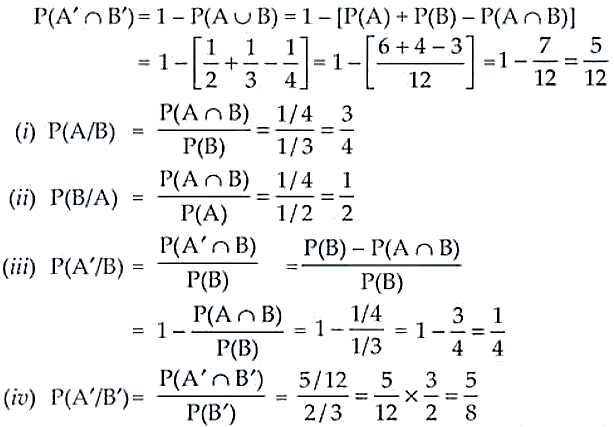8. Three events A, B and C have probabilities 2/5, 1/3 and ½ respectively. Given that P(AÇ C) = 1/5 and P(B Ç C) = ¼, find the values of P(C | B) and P(A’Ç C’).

Solution:

Given, P(A) = 2/5, P(B) = 1/3 and P(C) = ½

P(A Ç C) = 1/5 and P(B Ç C) = ¼

So, P(C/B) = P(B Ç C)/ P(B) = (¼)/ (1/3) = ¾

P(A’ Ç C’) = 1 – P(A ⋃ C)

= 1 – [P(A) + P(C) – P(A Ç C)]

= 1 – [2/5 + ½ – 1/5] = 1 – 7/10 = 3/10

Therefore, the required probabilities are ¾ and 3/10.

9. Let E1 and E2 be two independent events such that p(E1) = p1 and P(E2) = p2. Describe in words of the events whose probabilities are:

(i) p1 p2 (ii) (1–p1) p2 (iii) 1 – (1 – p1)(1 – p2) (iv) p1 + p2 – 2p1p2

Solution:

Here, P(E1) = p1 and P(E2) = p2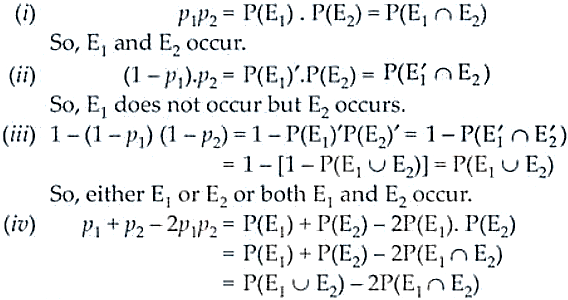Now, its clearly seen that either E1 or E2 occurs but not both.

10. A discrete random variable X has the probability distribution given as below:

 X 0.5 1 1.5 2 P(X) k k2 2k2 k

(i) Find the value of k

(ii) Determine the mean of the distribution.

Solution:

For a probability distribution, we know that if Pi ≥ 0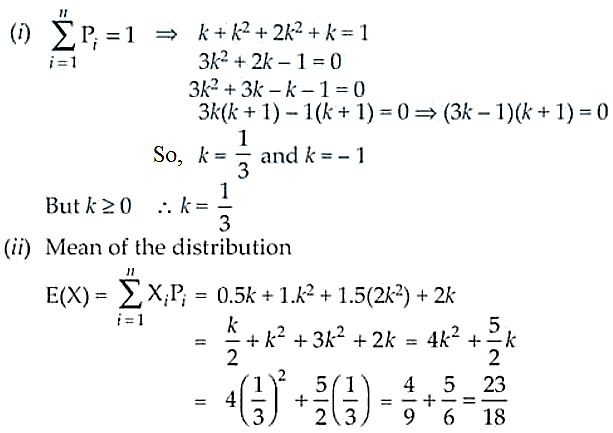11. Prove that

(i) P(A) = P(AÇ B) + P(AÇ B )

(ii) P(AÈ B) = P(AÇ B) + P(AÇ B ) + P( A Ç B)

Solution: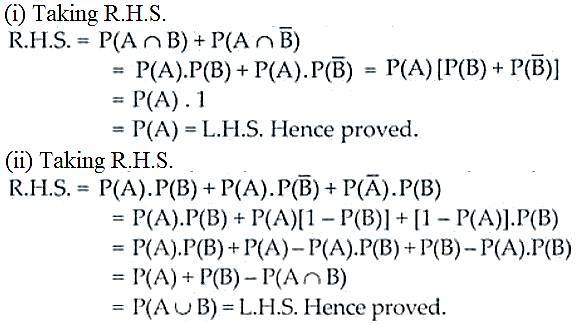12. If X is the number of tails in three tosses of a coin, determine the standard

deviation of X.

Solution:

Given, X = 0, 1, 2, 3

P(X = r) = nCr pr qn-r

Where n = 3, p = ½, q = ½ and r = 0, 1, 2, 3

P(X = 0) = ½ x ½ x ½ = 1/8

P(X = 1) = 3 x ½ x ½ x ½ = 3/8

P(X = 2) = 3 x ½ x ½ x ½ = 3/8

P(X = 3) = ½ x ½ x ½ = 3/8

The probability distribution table is:

 X 0 1 2 3 P(X) 1/8 3/8 3/8 1/8

Now,

E(X) = 0 + 1 x 3/8 + 2 x 3/8 + 3 x 1/8 = 3/8 + 6/8 + 3/8 = 12/8 = 3/2

E(X2) = 0 + 1 x 3/8 + 4 x 3/8 + 9 x 1/8 = 3/8 + 12/8 + 9/8 = 24/8 = 3

W.k.t, Var(X) = E(X2) – [E(X)]2 = 3 – (3/2)2 = 3 – (3/2)2 = 3 – 9/4 = ¾

Thus, the standard deviation = Var(X) = √(¾) = √3/2

13. In a dice game, a player pays a stake of Re1 for each throw of a die. She receives Rs 5 if the die shows a 3, Rs 2 if the die shows a 1 or 6, and nothing otherwise. What is the player’s expected profit per throw over a long series of throws?

Solution:

Let’s take X to be the random variable of profit per throw.

 X -1 1 4 P(X) 1/2 1/3 1/6

As, she loses Rs 1 for giving any od 2, 4, 5.

So, P(X = -1) = 1/6 + 1/6 + 1/6 = 3/6 = ½

P(X = 1) = 1/6 + 1/6 = 2/6 = 1/3 [Since, die showing 1 or 6]

P(X = 4) = 1/6 [Since, die shows only a 3]

Thus, the player’s expected profit = Ʃp1xi

= -1 x ½ + 1 x 1/3 + 4 x 1/6 = -1/2 + 1/3 + 2/3 = ½ = Rs 0.50

14. Three dice are thrown at the same time. Find the probability of getting three two’s, if it is known that the sum of the numbers on the dice was six.

Solution:

Given that the dice is thrown three times

So, the sample space n(S) = 63 = 216

Let E1 be the event when the sum of number on the dice was 6 and E2 be the event when three 2’s occur.

E1 = {(1, 1, 4), (1, 2, 3), (1, 4, 1), (2, 1, 3), (2, 2, 2), (2, 3, 1), (3, 1, 2), (3, 2, 1), (4, 1, 1)}

n(E1) =10 and n(E2) = 1 [Since, E2 = (2, 2, 2)]

Thus, P(E2/E1) = P(E1 ⋂ E2)/P(E1) = (1/216)/(10/216) = 1/10.

15. Suppose 10,000 tickets are sold in a lottery each for Re 1. First prize is of Rs 3000 and the second prize is of Rs. 2000. There are three third prizes of Rs. 500 each. If you buy one ticket, what is your expectation.

Solution:

Let’s take X to be the random variable where X = 0, 500, 2000 and 3000

 X 0 500 2000 3000 P(X) 9995/10000 3/10000 1/10000 1/10000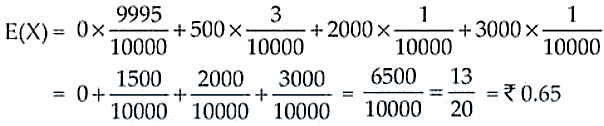16. A bag contains 4 white and 5 black balls. Another bag contains 9 white and 7 black balls. A ball is transferred from the first bag to the second and then a ball is drawn at random from the second bag. Find the probability that the ball drawn is white.

Solution:

Let’s take W1 and W2 to be two bags containing (4W, 5B) and (9W, 7B) balls respectively.

Let E1 be the event that the transferred ball from the bag W1 to W2 is white and E2 the event that the transferred ball is black.

And, E be the event that the ball drawn from the second bag is white.

So, the probabilities are:

P(E/E1) = 10/17, P(E/E2) = 9/17

P(E1) = 4/9 and P(E2) = 5/9

Now, P(E) = P(E1).P(E/E1) + P(E2).P(E/E2)

= 4/9 x 10/17 + 5/9 x 9/17 = 40/153 + 45/153 = 85/153 = 5/9

Therefore, the required probability is 5/9.

17. Bag I contains 3 black and 2 white balls, Bag II contains 2 black and 4 white balls. A bag and a ball is selected at random. Determine the probability of selecting a black ball.

Solution:

Given that:

Bag 1 has 3B, 2W balls and Bag 2 has 2B, 4W balls.

Let E1 = The event that bag 1 is selected

E2 = The event that bag 2 is selected

And, E = The event that a black ball is selected

Now, the probabilities are: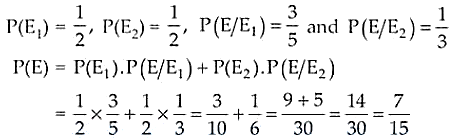Therefore, the required probability is 7/15.

18. A box has 5 blue and 4 red balls. One ball is drawn at random and not replaced. Its colour is also not noted. Then another ball is drawn at random. What is the probability of second ball being blue?

Solution:

Given that the box has 5 blue and 4 red balls.

Let’s consider E1 be the event that first ball drawn is blue and E2 be the event that first ball drawn is red.

And, E is the event that second ball drawn is blue.

Now, the probability of E is: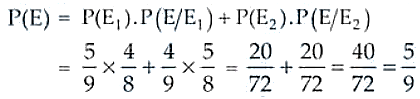Therefore, the required probability is 5/9.

19. Four cards are successively drawn without replacement from a deck of 52 playing cards. What is the probability that all the four cards are kings?

Solution:

Let E1, E2, E3 and E4 be the events that first, second, third and fourth card is King respectively.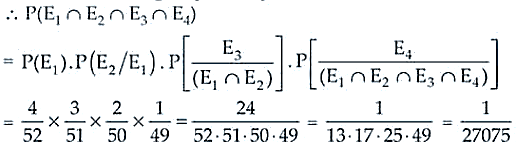Therefore, the required probability is 1/27075.

20. A die is thrown 5 times. Find the probability that an odd number will come up exactly three times.

Solution:

Here, p = 1/6 + 1/6 + 1/6 = ½ ⇒ q = 1 – ½ = ½ and n = 5

Now,

P(x = r) = nCr pr qn – r = 5C3 (1/2)3(1/2)5-3

= [5!/(3!2!)].(1/2)3(1/2)2 = 10.1/8.1/4 = 5/16

Therefore, the required probability is 5/16.

21. Ten coins are tossed. What is the probability of getting at least 8 heads?

Solution:

Here we have, n = 10, p = ½ and q = 1 – ½ = ½

P(X ≥ 8) = P(x = 8) + P(x = 9) + P(x = 10)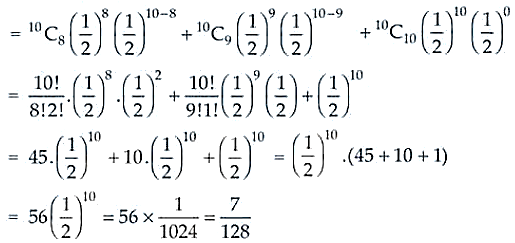Therefore, the required probability is 7/128.

22. The probability of a man hitting a target is 0.25. He shoots 7 times. What is the probability of his hitting at least twice?

Solution:

Here, we have n = 7, p = 0.25 = 25/100 = ¼ and q = 1 – ¼ = ¾

P(X ≥ 2) = 1 – [P(X = 0) + P(X = 1)]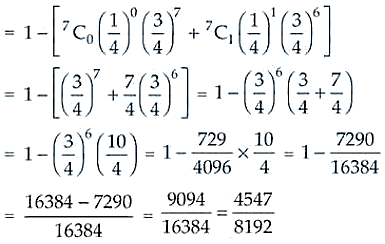Therefore, the required probability is 4547/8192.

23. A lot of 100 watches is known to have 10 defective watches. If 8 watches are selected (one by one with replacement) at random, what is the probability that there will be at least one defective watch?

Solution:

Given: Total number of watches = 100 and number of defective watches = 10

So, the probability of selecting a detective watch = 10/100 = 1/10

Now,

n = 8, p = 1/10 and q = 1 – 1/10 = 9/10, r ≥ 1

P(X ≥ 1) = 1 – P(x = 0) = 1 – 8C0 (1/10)0(9/10)8 – 0 = 1 – (9/10)8

Therefore, the required probability is 1 – (9/10)8.

24. Consider the probability distribution of a random variable X:

 X 0 1 2 3 4 P(X) 0.1 0.25 0.3 0.2 0.15

Calculate: (i) V(X/2) (ii) Variance of X.

Solution:

Given:

 X 0 1 2 3 4 P(X) 0.1 0.25 0.3 0.2 0.15

We know that: Var(X) = E(X2) – [E(X)]2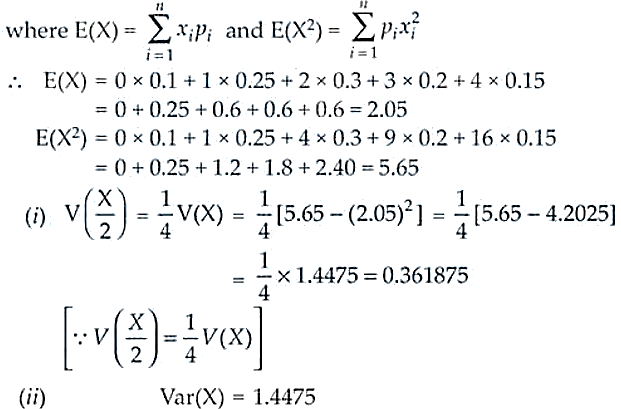25. The probability distribution of a random variable X is given below:

 X 0 1 2 3 P(X) k k/2 k/4 k/8

(i) Determine the value of k.

(ii) Determine P(X £ 2) and P(X > 2)

(iii) Find P(X £ 2) + P (X > 2).

Solution:

(i) W.k.t P(0) + P(1) + P(2) + P(3) = 1

⇒ k + k/2 + k/4 + k/8 = 1

(8k + 4k + 2k + k)/8 = 1

15k = 8

Hence, k = 8/15

(ii) P(X ≤ 2) = P(X = 0) + P(X = 1) + P(X = 2)

= k + k/2 + k/4 = 7k/4 = 7/4 x 8/15 = 14/15

And P(X > 2) = P(X = 3) = k/8 = 1/8 x 8/15 = 1/15

(iii) P(X ≤ 2) + P(X ≥ 2) = 14/15 + 1/15 = (14 + 1)/15 = 15/15 = 1

26. For the following probability distribution determine standard deviation of the random variable X.

 X 2 3 4 P(X) 0.2 0.5 0.3

Solution:

We know that: Standard deviation (S.D.) = √Variance

So, Var(X) = E(X2) – [E(X)]2

E(X) = 2 x 0.2 + 3 x 0.5 + 4 x 0.3 = 0.4 + 1.5 + 1.2 = 3.1

E(X2) = 4 x 0.2 + 9 x 0.5 + 16 x 0.3 = 0.8 + 4.5 + 4.8 = 10.1

⇒ V(X) = 10.1 – (3.1)2 = 10.1 – 9.61 = 0.49

Therefore, S.D. = √0.49 = 0.7

27. A biased die is such that P(4) = 1/10 and other scores being equally likely. The die

is tossed twice. If X is the ‘number of fours seen’, find the variance of the

random variable X.

Solution:

Here, random variable X = 0, 1, 2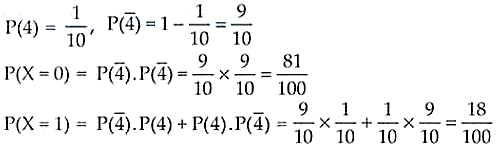P(X = 2) = P(4).P(4) = 1/10 x 1/10 = 1/100

 X 0 1 2 P(X) 81/100 18/100 1/100

Now, we know V(X) = E(X2) – [E(X)]2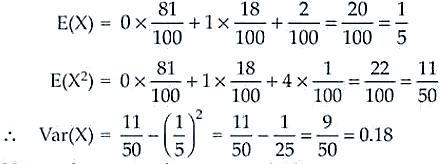Therefore, the required variance = 0.18.

28. A die is thrown three times. Let X be ‘the number of twos seen’. Find the

expectation of X.

Solution:

Here, we have X = 0, 1, 2, 3 [Since, die is thrown 3 times]

And p = 1/6, q = 5/6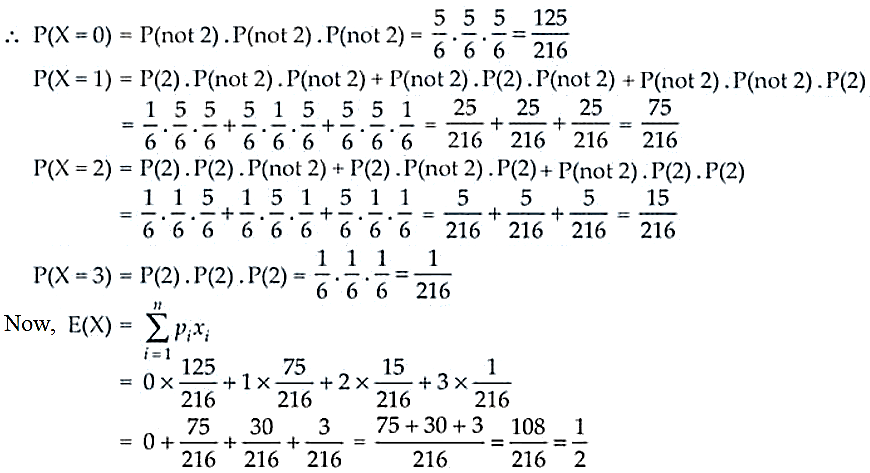Therefore, the required expression is ½.

29. Two biased dice are thrown together. For the first die P(6) = 1/2, the other scores being equally likely while for the second die, P(1) = 2/5 and the other scores are equally likely. Find the probability distribution of ‘the number of ones seen’.

Solution: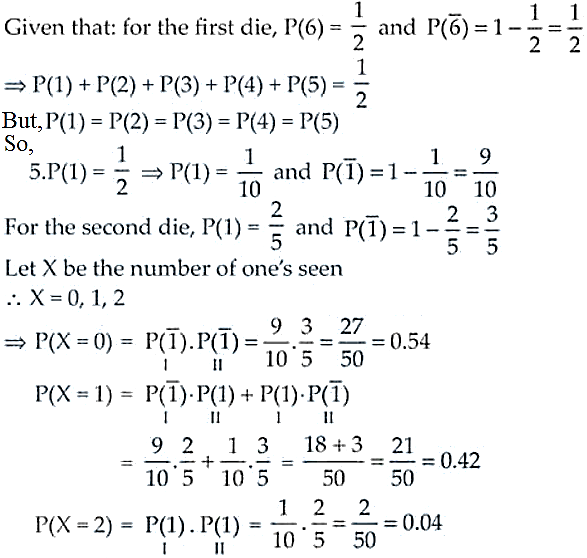Therefore, the required probability distribution is

 X 0 1 2 P(X) 0.54 0.42 0.04

30. Two probability distributions of the discrete random variable X and Y are given below.

 X 0 1 2 3 P(X) 1/5 2/5 1/5 1/5 Y 0 1 2 3 P(Y) 1/5 3/10 2/5 1/10

Prove that E(Y2) = 2 E(X).

Solution:

The probability distribution of random variable X is

 X 0 1 2 3 P(X) 1/5 2/5 1/5 1/5

We know that,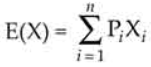E(X) 0. 1/5 + 1. 2/5 + 2. 1/5 + 3. 1/5 = 0 + 2/5 + 2/5 + 3/5 = 7/5

For the second probability distribution of random variable Y,

 Y 0 1 2 3 P(Y) 1/5 3/10 2/5 1/10

E(Y2) = 0. 1/5 + 1. 3/10 + 4. 2/5 + 9. 1/10

= 0 + 3/10 + 8/5 + 9/10 = 28/10 = 14/5

Now, E(Y2) = 14/5 and 2 E(X) = 2.7/5 = 14/5

Therefore, E(Y2) = 2E(X)

31. A factory produces bulbs. The probability that any one bulb is defective is 1/50 and they are packed in boxes of 10. From a single box, find the probability that

(i) none of the bulbs is defective

(ii) exactly two bulbs are defective

(iii) more than 8 bulbs work properly

Solution:

Let’s assume X to be the random variable denoting a bulb to be defective.

Here, n = 10, p = 1/50, q = 1 – 1/50 = 49/50

We know that, P(X = r) = nCr pr qn – r

(i) None of the bulbs is defective, i.e., r = 0

P(x = 0) = 10C0 (1/50)0(49/50)10 – 0 = (49/50)10

(ii) Exactly two bulbs are defective

So, P(x = 2) = 10C2 (1/50)2(49/50)10 – 2

= 45.498/5010 = 45 x (1/50)10 x 498

(iii) More than 8 bulbs work properly

We can say that less than 2 bulbs are defective

P(x < 2) = P(x = 0) + P(x = 1)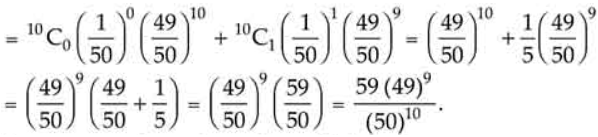32. Suppose you have two coins which appear identical in your pocket. You know that one is fair and one is 2-headed. If you take one out, toss it and get a head, what is the probability that it was a fair coin?

Solution:

Let’s consider

E1 = Event that the coin is fair

E2 = Event that the coin is 2 headed

And H = Event that the tossed coin gets head.

Now,

P(E1) = ½, P(E2) = ½, P(H/E1) = ½, P(H/E2) = 1

Using Baye’s Theorem, we get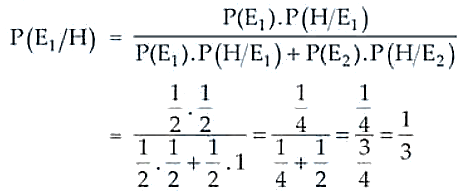Therefore, the required probability is 1/3.

33. Suppose that 6% of the people with blood group O are left-handed and 10% of those with other blood groups are left-handed 30% of the people have blood group O. If a left-handed person is selected at random, what is the probability that he/she will have blood group O?

Solution:

Let’s assume

E1 = The event that a person selected is of blood group O

E2 = The event that the people selected is of other group

And H = The event that selected person is left handed

Now,

P(E1) = 0.30 and P(E2) = 0.70

Further, P(H/E1) = 0.06 and P(H/E2) = 0.10

Using Baye’s Theorem, we have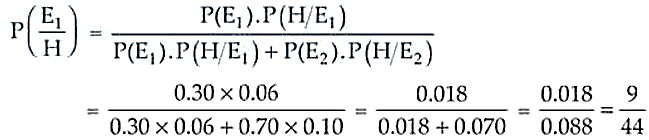Therefore, the required probability is 9/44.

34. Two natural numbers r, s are drawn one at a time, without replacement from the set S={1, 2, 3, …., n} . Find P[r £ p|s £ p] , where pÎS.

Solution:

Given, S = {1, 2, 3, …, n}

So, P(r ≤ p/s ≤ p) = P(P ⋂ S)/ P(S)

= p – 1/n x n/(n – 1)

= (p – 1)/(n – 1)

Therefore, the required probability is (p – 1)/(n – 1).

35. Find the probability distribution of the maximum of the two scores obtained when a die is thrown twice. Determine also the mean of the distribution.

Solution:

Let X be the random variable scores when a die is thrown twice.

X = 1, 2, 3, 4, 5, 6

And S = {(1, 1), (1, 2), (2, 1), (2, 2) , (1, 3) , (2, 3) , (3, 1) , (3, 2) , (3, 3) , (3, 4) , (3, 5) , …, (6, 6)}

So,

P(X = 1) = 1/6 . 1/6 = 1/36

P(X = 2) = 1/6 . 1/6 + 1/6 . 1/6 + 1/6 . 1/6 = 3/36

P(X = 3) = 1/6 . 1/6 + 1/6 . 1/6 + 1/6 . 1/6 + 1/6 . 1/6 + 1/6 . 1/6 = 5/36

Similarly,

P(X = 4) = 7/36; P(X = 5) = 9/36 and P(X = 6) = 11/36

Hence, the required distribution is

 X 1 2 3 4 5 6 P(X) 1/36 3/36 5/36 7/36 9/36 11/36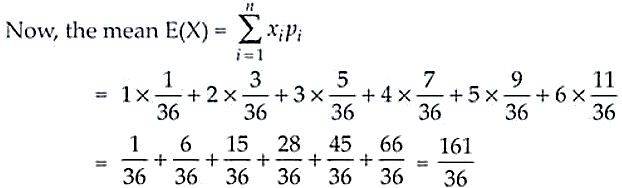Therefore, the required mean = 161/36.

36. The random variable X can take only the values 0, 1, 2. Given that P(X = 0) = P (X = 1) = p and that E(X2) = E[X], find the value of p.

Solution:

Given, X = 0, 1, 2 and P(X = 0) = P (X = 1) = p

Let P(X) at X = 2 is x

⇒ p + p + x = 1 ⇒ x = 1 – 2p

Now, we have the following distribution:

 X 0 1 2 P(X) p p 1 – 2p

So,

E(X) = 0.p + 1.p + 2(1 – 2p) = p + 2 – 4p = 2 – 3p

And, E(X2) = 0.p + 1.p + 4(1 – 2p) = p + 4 – 8p = 4 – 7p

Also, given E(X) = E(X2)

2 – 3p = 4 – 7p

4p = 2

p = ½

Therefore, the required value of p is ½.

37. Find the variance of the distribution:

 x 0 1 2 3 4 5 P(x) 1/6 5/18 2/9 1/6 1/9 1/18

Solution:

We know that,

Variance(X) = E(X2) – [E(X)]2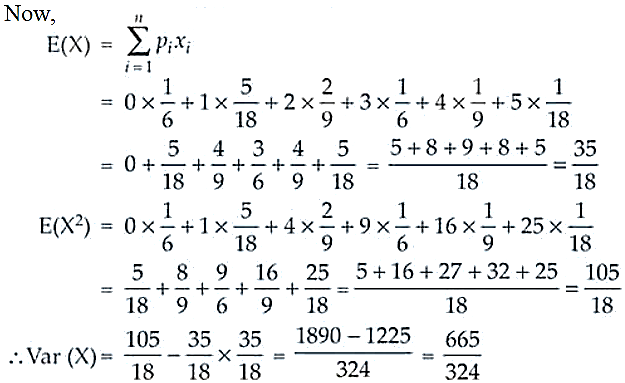Therefore, the required variance is 665/324.

38. A and B throw a pair of dice alternately. A wins the game if he gets a total of 6 and B wins if she gets a total of 7. It A starts the game, find the probability of winning the game by A in third throw of the pair of dice.

Solution:

Let’s take A1 to be the event of getting a total of 6

A1 = {(2, 4), (4, 2), (1, 5), (5, 1), (3, 3)}

And, B1 be the event of getting a total of 7

B1 = {(2, 5), (5, 2), (1, 6), (6, 1), (3, 4), (4, 3)}

Let P(A1) is the probability, if A wins in a throw = 5/36

And P(B1) is the probability, if B wins in a throw = 1/6

Therefore, the required probability of wining A in his third throw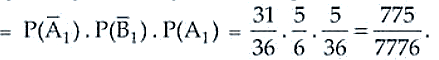39. Two dice are tossed. Find whether the following two events A and B are independent:

A = {(x, y) : x + y = 11} B = {(x, y) : x ¹ 5}

where (x, y) denotes a typical sample point.

Solution:

Given, two events A and B are independent such that A = {(x, y) : x + y = 11} B = {(x, y) : x ¹ 5}

Now,

A = {(5, 6), (6, 5)}

B = {(1, 1), (1, 2), (1, 3), (1, 5), (1, 6), (2, 1), (2, 2), (2, 4), (2, 5), (2, 6), (3, 1), (3, 3), (3, 4), (3, 5), (3, 6), (4, 2), (4, 3), (4, 4), (4, 5), (4, 6), (5, 1), (5, 2), (5, 3), (5, 4), (5, 5), (5, 6), (6, 1), (6, 2), (6, 3), (6, 4), (6, 5), (6, 6)}

n(A) = 2, n(B) = 30 and n(A ⋂ B) = 1

So, P(A) = 2/36 = 1/18 and P(B) = 30/36 = 5/6

Now,

P(A).P(B) = 1/18. 5/6 = 5/108 and P(A ⋂ B) 1/36

As P(A). P(B) ≠ P(A ⋂ B)

Therefore, events A and B are not independent.

40. An urn contains m white and n black balls. A ball is drawn at random and is put back into the urn along with k additional balls of the same colour as that of the ball drawn. A ball is again drawn at random. Show that the probability of drawing a white ball now does not depend on k.

Solution:

Let’s consider A to be the event of having m white and n black balls

E1 = First ball drawn of white colour

E2 = First ball drawn of black colour

E3 = Second ball drawn of white colour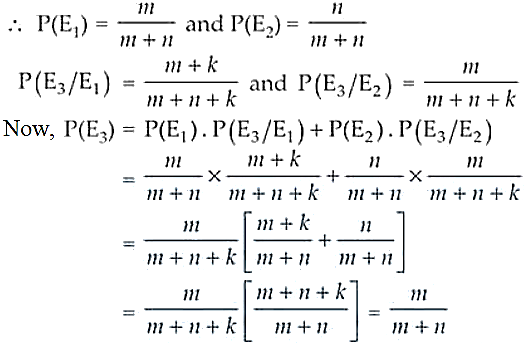Therefore, the probability if drawing a white ball does not depend upon k.

41. Three bags contain a number of red and white balls as follows:

Bag 1 : 3 red balls, Bag 2 : 2 red balls and 1 white ball

Bag 3 : 3 white balls.

The probability that bag i will be chosen and a ball is selected from it is i/6, i = 1, 2, 3. What is the probability that

(i) a red ball will be selected? (ii) a white ball is selected?

Solution:

Given:

Bag 1 : 3 red balls,

Bag 2 : 2 red balls and 1 white ball

Bag 3 : 3 white balls

Now, let E1, E2 and E3 be the events of choosing Bag 1, Bag 2 and Bag 3 respectively and a ball is drawn from it.

And, we have

P(E1) = 1/6, P(E2) = 2/6 and P(E3) = 3/6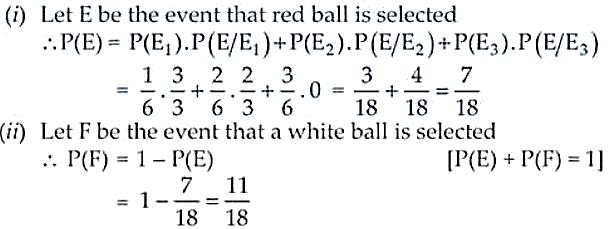Therefore, the required probabilities are 7/18 and 11/18.

42. Refer to Question 41 above. If a white ball is selected, what is the probability

that it came from (i) Bag 2 (ii) Bag 3

Solution:

Referring the Question 41, here we will use Baye’s Theorem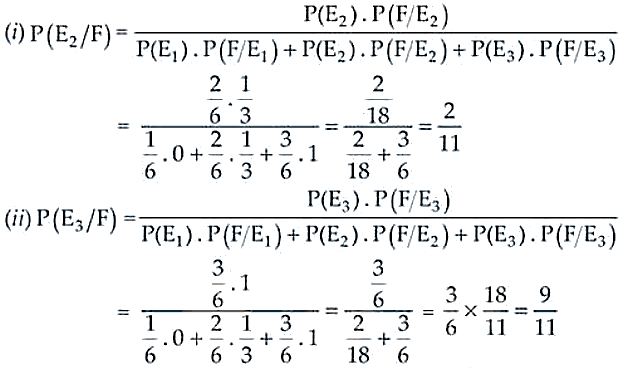Therefore, the required probabilities are 2/11 and 9/11.

43. A shopkeeper sells three types of flower seeds A1, A2 and A3. They are sold as a mixture where the proportions are 4:4:2 respectively. The germination rates of the three types of seeds are 45%, 60% and 35%. Calculate the probability

(i) of a randomly chosen seed to germinate

(ii) that it will not germinate given that the seed is of type A3,

(iii) that it is of the type A2 given that a randomly chosen seed does not germinate.

Solution:

Given that: A1: A2: A3 = 4: 4: 2

So, the probabilities will be

P(A1) = 4/10, P(A2) = 4/10 and P(A3) = 2/10,

Where A1, A2 and A3 are the three types of seeds.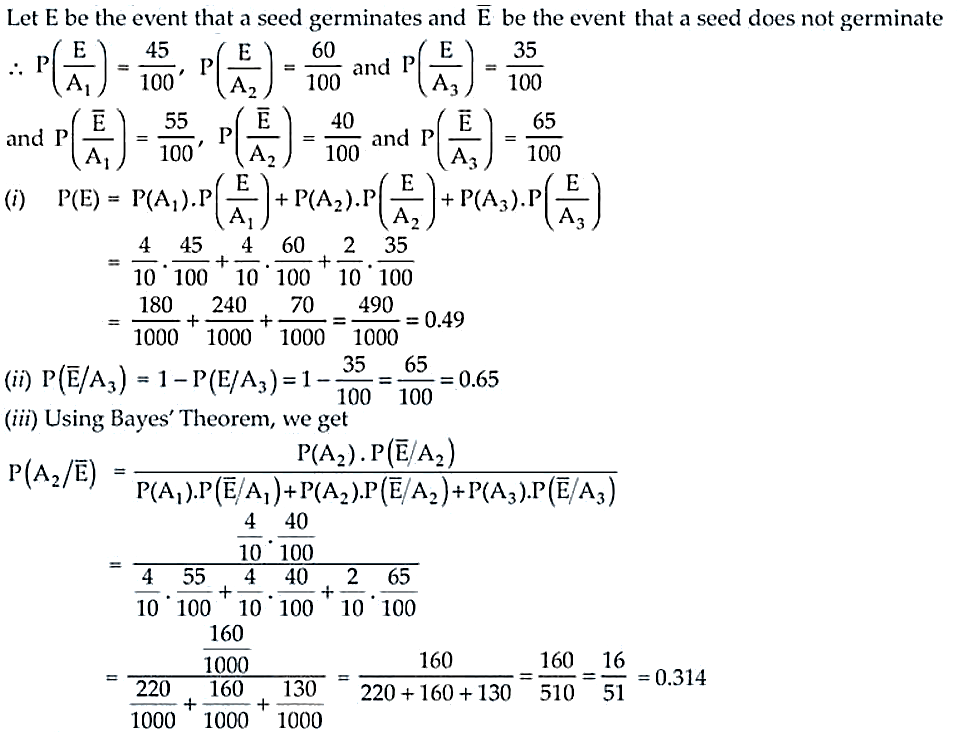Therefore, the required probability is 16/51 or 0.314.

44. A letter is known to have come either from TATA NAGAR or from CALCUTTA. On the envelope, just two consecutive letter TA are visible. What is the probability that the letter came from TATA NAGAR.

Solution:

Let E1 be the event that the letter comes from TATA NAGAR,

E2 be the event that the letter comes from CALCUTTA

And, E3 be the event that on the letter, two consecutive letters TA are visible

Now,

P(E1) = ½, P(E2) = ½, P(E3/E1) = 2/8 and P(E3/E2) = 1/7

For TATA NAGAR, the two consecutive letters visible are TA, AT, TA, AN, NA, AG, GA and AR

So, P(E3/E1) = 2/8

And, for CALCULTTA the two consecutive letters visible are CA, AL, LC, CU, UT, TT and TA

So, P(E3/E2) = 1/7

Now, using Baye’s Theorem we haveTherefore, the required probability is 7/11.

45. There are two bags, one of which contains 3 black and 4 white balls while the other contains 4 black and 3 white balls. A die is thrown. If it shows up 1 or 3, a ball is taken from the Ist bag; but it shows up any other number, a ball is chosen from the second bag. Find the probability of choosing a black ball.

Solution:

Let E1 be the event of selecting Bag 1 and E2 be the event of selecting Bag 2.

Also, let E3 be the event that black ball is selected

Now,

P(E1) = 2/6 = 1/3 and P(E2) = 1 – 1/3 = 2/3

P(E3/E1) = 3/7 and P(E3/E2) = 4/7

So,

P(E3) = P(E1). P(E3/E1) + P(E2). P(E3/E2)

= 1/3. 3/7 + 2/3. 4/7 = (3 + 8)/ 21 = 11/21

Therefore, the required probability is 11/21.

46. There are three urns containing 2 white and 3 black balls, 3 white and 2 black balls, and 4 white and 1 black balls, respectively. There is an equal probability of each urn being chosen. A ball is drawn at random from the chosen urn and it is found to be white. Find the probability that the ball drawn was from the second urn.

Solution:

Given, we have 3 urns:

Urn 1 = 2 white and 3 black balls

Urn 2 = 3 white and 2 black balls

Urn 3 = 4 white and 1 black balls

Now, the probabilities of choosing either of the urns are

P(U1) = P(U2) = P(U3) = 1/3

Let H be the event of drawing white ball from the chosen urn.

So,

P(H/U1) = 2/5, P(H/U2) = 3/5 and P(H/U3) = 4/5

By using Baye’s Theorem, we have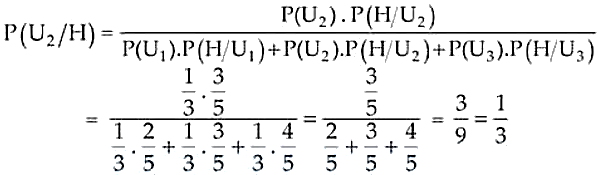Therefore, the required probability is 1/3.

47. By examining the chest X ray, the probability that TB is detected when a person is actually suffering is 0.99. The probability of an healthy person diagnosed to have TB is 0.001. In a certain city, 1 in 1000 people suffers from TB. A person is selected at random and is diagnosed to have TB. What is the probability that he actually has TB?

Solution:

Let E1 = Event that a person has TB

E2 = Event that a person does not have TB

And H = Event that the person is diagnosed to have TB.

So,

P(E1) = 1/1000 = 0.001, P(E2) = 1 – 1/1000 = 999/1000 = 0.999

P(H/E1) = 0.99, P(H/E2) = 0.001

Now, using Baye’s theorem we have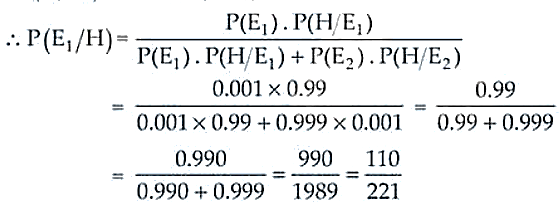Therefore, the required probability is 110/221.

48. An item is manufactured by three machines A, B and C. Out of the total number of items manufactured during a specified period, 50% are manufactured on A, 30% on B and 20% on C. 2% of the items produced on A and 2% of items produced on B are defective, and 3% of these produced on C are defective. All the items are stored at one godown. One item is drawn at random and is found to be defective. What is the probability that it was manufactured on machine A?

Solution:

Let’s consider:

E1 = The event that the item is manufactured on machine A

E2 = The event that the item is manufactured on machine B

E3 = The event that the item is manufactured on machine C

Let H be the event that the selected item is defective.

Thus, by using Baye’s theorem we have

P(E1) = 50/100, P(E2) = 30/100, P(E3) = 20/100

P(H/E1) = 2/100, P(H/E2) = 2/100 and P(H/E3) = 3/100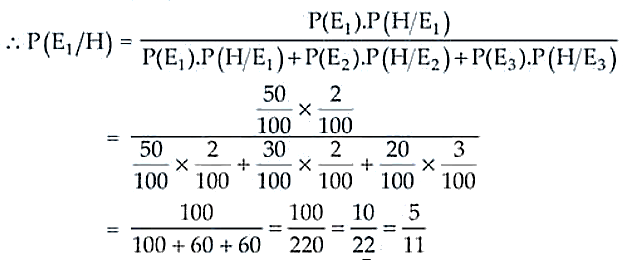Therefore, the required probability is 5/11.

49. Let X be a discrete random variable whose probability distribution is defined as follows: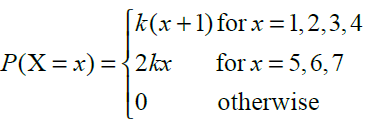where k is a constant. Calculate

(i) the value of k (ii) E (X) (iii) Standard deviation of X.

Solution:

(i) Given, P(X = x) = k(x + 1) for x = 1, 2, 3, 4

So, P(X = 1) = k(1 + 1) = 2k

P(X = 2) = k(2 + 1) = 3k

P(X = 3) = k(3 + 1) = 4k

P(X = 4) = k(4 + 1) = 5k

Also, P(X = x) = 2kx for x = 5, 6, 7

P(X = 5) = 2k(5) = 10k

P(X = 6) = 2k(6) = 12k

P(X = 7) = 2k(7) = 14k

And, for otherwise it is 0.

Thus, the probability distribution is given by

 X 1 2 3 4 5 6 7 Otherwise P(X) 2k 3k 4k 5k 10k 12k 14k 0

We know that,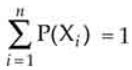So, 2k + 3k + 4k + 5k + 10k + 12k + 14k = 1

⇒ 50k = 1

⇒ k = 1/50

Therefore, the value of k is 1/50.

(ii) Now, the probability distribution is

 X 1 2 3 4 5 6 7 P(X) 2/50 3/50 4/50 5/50 10/50 12/50 14/50

E(X) = 1 x 2/50 + 2 x 3/50 + 3 x 4/50 + 4 x 5/50 + 5 x 10/50 + 6 x 12/50 + 7 x 14/50

= 2/50 + 6/50 + 12/50 + 20/50 + 50/50 + 72/50 + 98/50

= 260/50

= 26/5 = 5.2

(ii) We know that the standard deviation (SD) = √Variance

Variance = E(X2) – [E(X)]2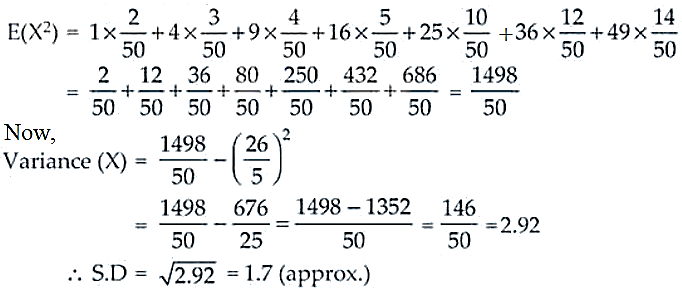50. The probability distribution of a discrete random variable X is given as under:

 X 1 2 4 2A 3A 5A P(X) 1/2 1/5 3/25 1/10 1/25 1/25

Calculate:

(i) The value of A if E(X) = 2.94

(ii) Variance of X.

Solution:

(i) We know that: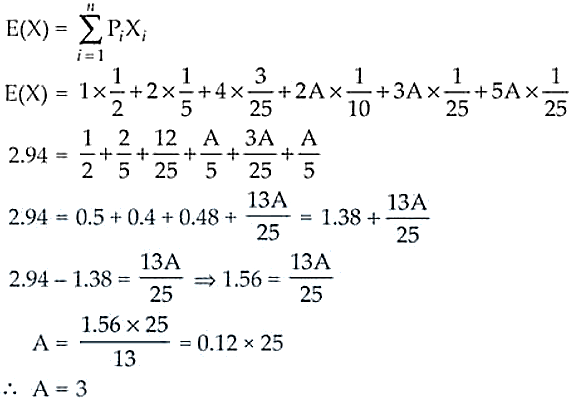(ii) Now, the distribution becomes

 X 1 2 4 6 9 15 P(X) 1/2 1/5 3/25 1/10 1/25 1/25

E(X2) = 1 x ½ + 4 x 1/5 + 16 x 3/25 + 36 x 1/10 + 81 x 1/25 + 225 x 1/25

= ½ + 4/5 + 48/25 + 36/10 + 81/25 + 225/25

= 0.5 + 0.8 + 1.92 + 3.6 + 3.24 + 9 = 19.06

Variance (X) = E(X2) – [E(X)]2

= 19.06 – (2.94)2 = 19.06 – 8.64 = 10.42

51. The probability distribution of a random variable x is given as under: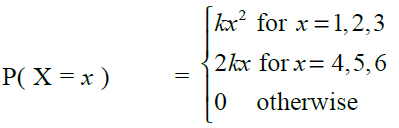where k is a constant. Calculate

(i) E(X) (ii) E (3X2) (iii) P(X ³ 4)

Solution:

The probability distribution of random variable X is given by:

 X 1 2 3 4 5 6 Otherwise P(X) k 4k 9k 8k 10k 12k 0

We know that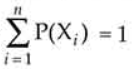So, k + 4k + 9k + 8k + 10k + 12k = 1

44k = 1k = 1/44

(i) E(X) =

= 1 x k + 2 x 4k + 3 x 9k + 4 x 8k + 5 x 10k + 6 x 12k

= k + 8k + 27k + 32k + 50k + 72k = 190k

= 190 x 1/44 = 95/22 = 4.32 (approx.)

(ii) E(X2) = 3(k + 4 x 4k + 9 x 9k + 16 x 8k + 25 x 10k + 36 x 12k)

= 3(k + 16k + 81k + 128k + 250k + 432k) = 3(908k)

= 3 x 908 x 1/44 = 2724/44 = 61.9 (approx.)

(iii) P(X ≥ 4) = P(X = 4) + P(X = 5) + P(X = 6)

= 8k + 10k + 12k = 30k

= 30 x 1/44 = 15/22

52. A bag contains (2n + 1) coins. It is known that n of these coins has a head on both sides whereas the rest of the coins are fair. A coin is picked up at random from the bag and is tossed. If the probability that the toss results in a head is 31/42, determine the value of n.

Solution:

Given, n coins are two headed coins and the remaining (n + 1) coins are fair.

Let E1: the event that unfair coin is selected

E2: the event that the fair coin is selected

E: the event that the toss results in a head

So,

P(E1) = n/(2n + 1) and P(E2) = (n + 1)/ (2n +1)

P(E/E1) = 1 (As it’s a sure event)

P(E/E2) = ½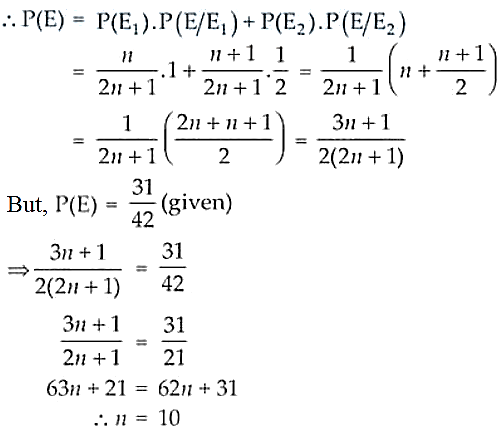Therefore, the required value of n is 10.

53. Two cards are drawn successively without replacement from a well shuffled deck of cards. Find the mean and standard variation of the random variable X where X is the number of aces.

Solution:

Let’s consider X to be the random variable such that X = 0, 1, 2

Now, let E = the event of drawing an ace

And, F = the event of drawing non – ace

So,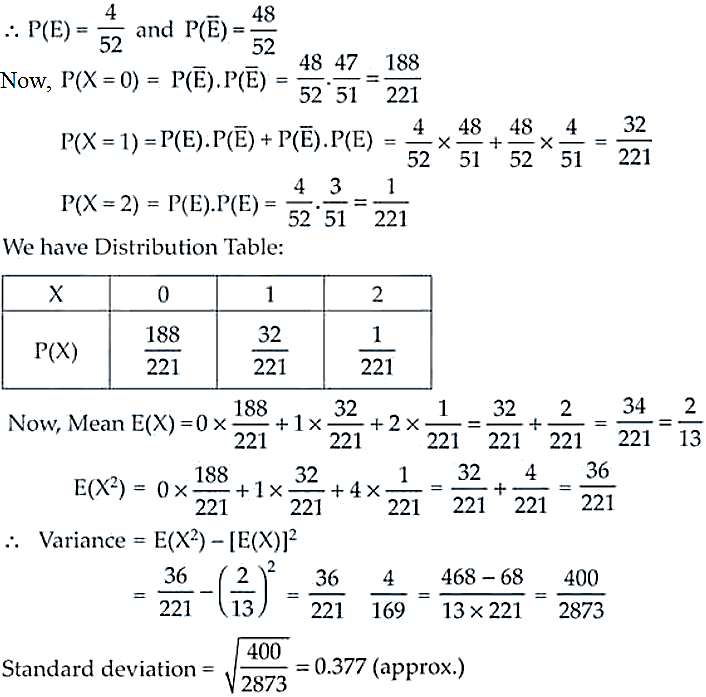54. A die is tossed twice. A ‘success’ is getting an even number on a toss. Find the variance of the number of successes.

Solution:

Let’s consider E to be the event of getting even number on tossing a die.